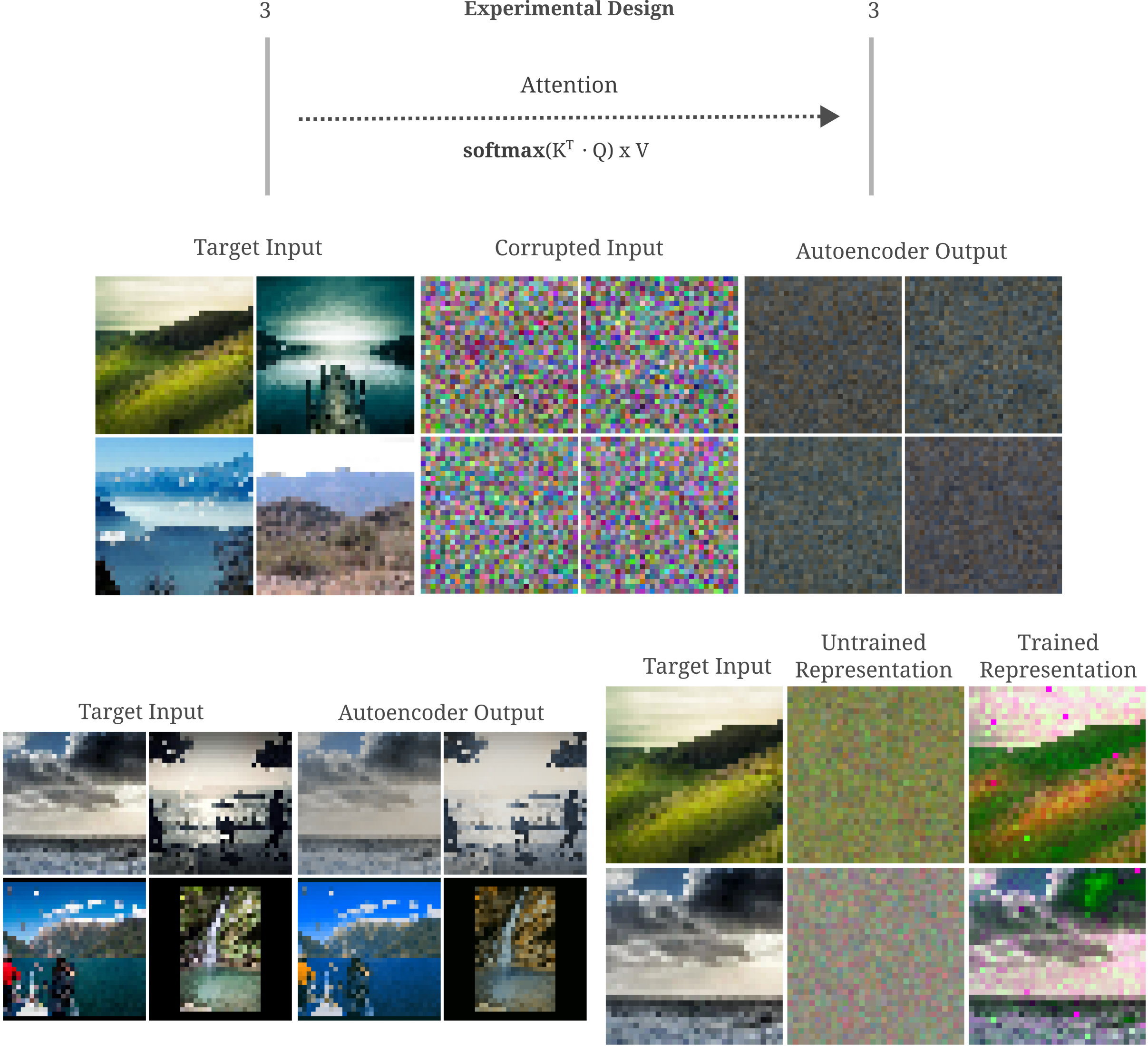## Autoencoders: Learning by Copying

### Introduction

Autoencoders (self-encoders) are machine learning models that attempt to replicate the input in their output. This endeavor may not seem to be very useful for tasks such as generating images or learning how inputs are related to one another, particularly when the models used are very large and fully capable of learning the identity function on the input. Indeed, in the past it was assumed that models capable of learning the identity function (typically models with more neurons per layer than input elements) would do so without other forms of regularization.

Historically, therefore, autoencoders were studied in two contexts: as undercomplete models that compressed the input in some useful way, and as possibly overcomplete models that were subject to certain restrictions to as to prevent the learning of the identity function. Here ‘overcomplete’ is used more informally than the strict linear algebra term, and signifies a model that has more than enough elements (neurons) per layer to exactly copy the input onto that layer.

A number of useful models have resulted from those efforts, including the contractive autoencoder which places a penalty on the norm of the Jacobian of the encoder with respect to the input, and the variational autoencoder which simultaneously seeks to minimize the difference between autoencoder output and input together with the difference between the hidden layer code $z$ given model inputs and a Gaussian distribution $N(z; I)$.

The primary drawback of these regularized autoencoders is that they tend to be incapable of modeling the distribution of inputs given to the model to a sufficient degree, which manifests experimentally as blurriness in samples drawn from these models. For variational autoencoders, there is a clear reason why samples would be blurry: transforming a typical image using a Gaussian distribution (via convolution or a similar method) results in a blurry copy of that image, so it is little surprise that enforcing the encoding of an input to approximate a Gaussian distribution would have the same effect. As a consequence of this challenge, much research on generative models (particularly for images) has shifted away to generative adversarial networks and more recently diffusion models.

Recently, however, two findings have brought the original assumption that overcomplete autoencoders would memorize their inputs into question. First, it has been observed that deep learning models for image classification tend to lose a substantial amount of input information by the time the input has been passed to deep layers, and that trained models learn to infer this lost information (reference). Second it has been found that image classification models are more or less guaranteed to generalize to some extent when trained using gradient descent (reference).

In light of these findings, it is worth asking whether overcomplete deep learning autoencoders will necessarily memorize their inputs. The answer to this question is straightforward, as we will see in the next section.

### Overcomplete autoencoders do not learn the identity function

The question of whether overcomplete autoencoders memorize their inputs can easily be tested by first training an autoencoder to replicate inputs, and then observing the tendancy of that autoencoder to copy its inputs after small changes are made. Autoencoders may be trained in various ways, and we choose to employ the minimization of Mean-Squared Error between the input $a$ and reconstructed input $D(E(a))$ where $D$ signifies the decoder of hidden code $h$, $E$ the encoder which maps $a \to h$ and $O(a, \theta)$ the output of the encoder and decoder given model parameters $\theta$.

$L(O(a, \theta), a) = || D(E(a)) - a ||^2_2$

Mean-squared error loss in this case is equivalent to the cross entropy between the model’s outputs and a Gaussian mixture model of those outputs. More precisely assuming that the distribution of targets $y$ given inputs $a$ is Gaussian, the likelihood of $y$ given input $a$ is

$p(y | a) = \mathcal{N}(y; \mu (O(a, \theta)), \sigma)$

where $\mu (O(a, \theta))$ signifies the mean of the output and $y=a$ for an autoencoder.

As for the case observed with variational autoencoders, it has been assumed that training autoencoders via MSE loss results in blurry output images because it implies that the input can be modelled by a Gaussian mixture model. And for a sufficiently small model this is true, being that only a small number of Gaussian distributions may compose the mixture model.

But for a very large model it is not the case that MSE loss (or any maximum likelihood estimation approach) will necessarily yield blurry outputs because the Gaussian mixture model is a universal approximator of computable functions if given enough individual Gaussian distributions independent from each other given weights $w_1, w_2, … w_n$

$g(a; w, \mu, \sigma) = \sum_i w_i \mathcal{N}(a; \mu_i, \sigma_i)$

Therefore it can be theoretically guaranteed that a sufficiently large autoencoder trained using MSE loss on the output alone will be capable of arbitrarily good approximations on a given input $a$ (and will not yield blurry samples).

This being the case, we can now test whether an overcomplete autoencoder trained using gradient descent to minimize MSE loss will memorize their inputs. Memorization in this case is equivalent to learning the identity function, so we can test memorization by simply changing an input some small amount before observing the output. If the identity function has been learned then the model will simply yield the same input back again.

One such change to an input is the addition of noise. In the following experiment we train a 5-hidden layer feedforward model, each layer having more elements that the input. As shown in the figure below, we find that indeed the overcomplete autoencoder does not memorize its inputs: upon the addition of Gaussian noise $\mathcal N(a \in [0, 1]; \mu=7/10, \sigma=2/10)$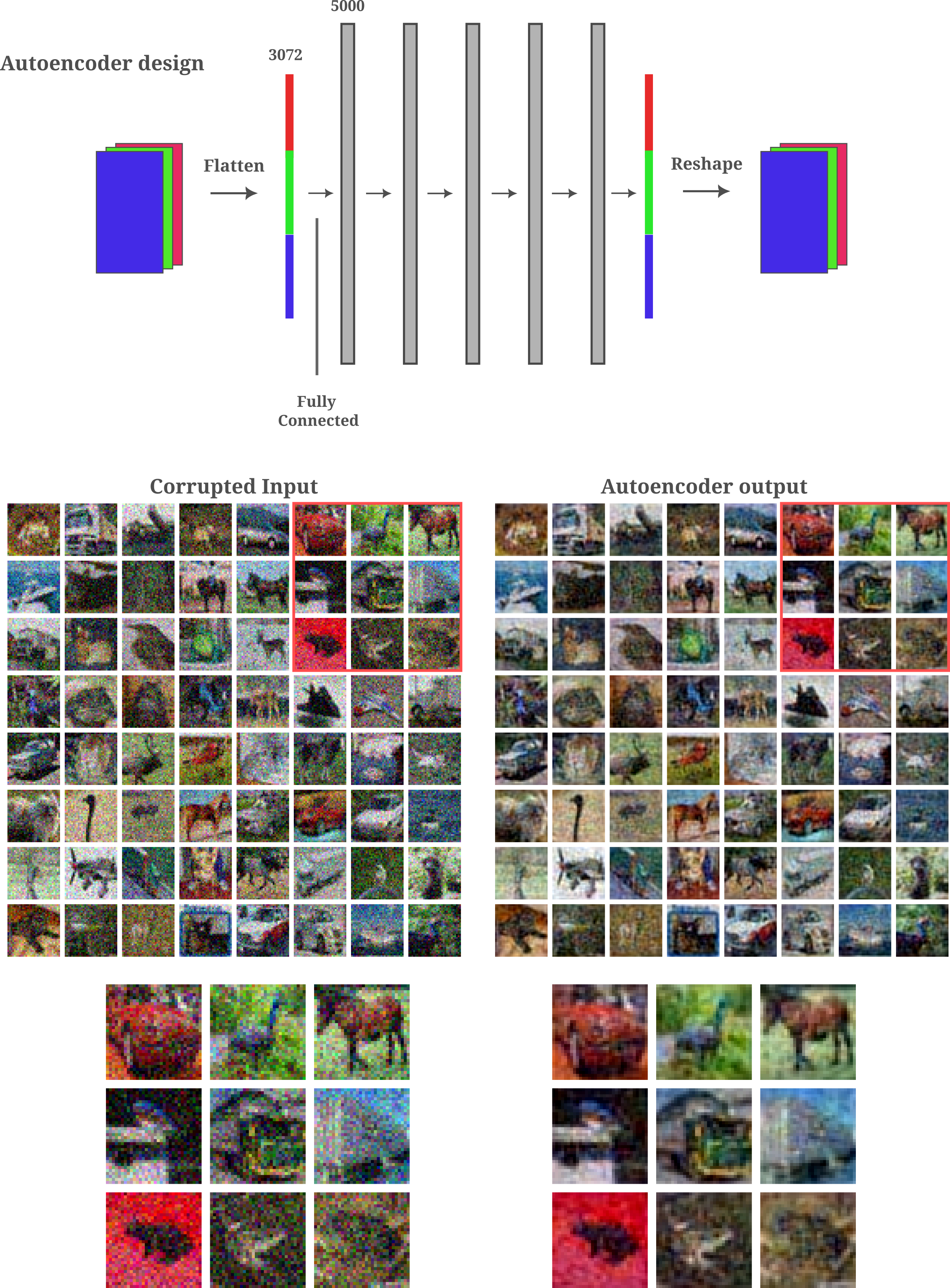This experiment provides evidence for two ideas: first that overcomplete autoencoders do not tend to learn the identity function as evidenced by the difference in the autoencoder’s output given a noise-corrupted input, $O(\widehat a, \theta)$ compared to the original input $a$, and second that the output actually de-noises the input such that

$||O(\widehat a, \theta) - a|| < || \widehat a - a ||$

The latter observation is far from trivial: there is no guarantee that a function learned by an autoencoder, even if this function is not the identity, would be capable of de-noising an input. We next investigate why this would occur.

### Autoencoders are capable of denoising without being trained to do so

To re-iterate, elsewhere it was found that deep learning models lose a substantial amount of information about the input by the time the forward pass reaches deeper layers, and furthermore that moels tend to learn to infer that lost information upon training. The learning process results in arbitrary inputs being mapped to the learned manifold, which for datasets of natural images should not normally be approximated by Gaussian noise. The learning process for natural image classifiers is therefore observed empirically to be as analagous to learning how to de-noise an input. One may safely assume that the same de-noising would occur upon training an autoencoder.

For this section and much of the rest of this page, we will employ a more sophistocated autoencoder than the one used above. We use U-net, a model introduced by Ronneburger and colleagues for medical image segmentation.

The U-net was named after it’s distinctive architecture,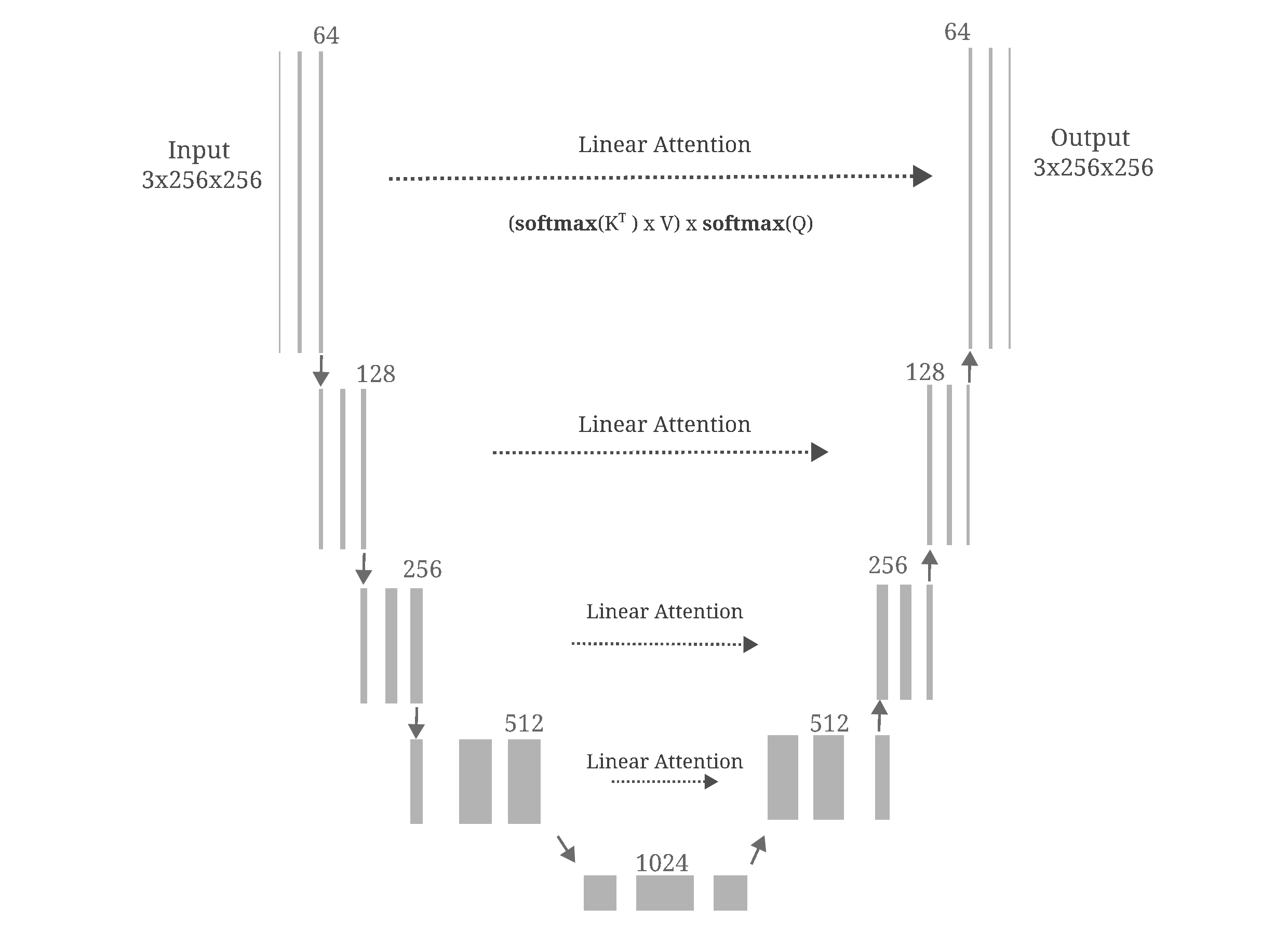For now, we make a few small adjustments to the Unet model: first we remove residual connections (which if not removed do lead to a model learning the identity function because it is effectively low-dimensional) and then we modify the stride of the upsampling convolutions to allow for increased resolution (code is available here). Notably we perform both training and sampling without switching to evaluation mode, such that the batch normalization transformations do not switch the batch’s statistics with averages accumulated during training.

Returning to the question of the tendancy of training to reduce noise in an autoencoder, take the last layer’s representations of the input in trained versus untrained Unet model (without residual layers) shown in the following figure.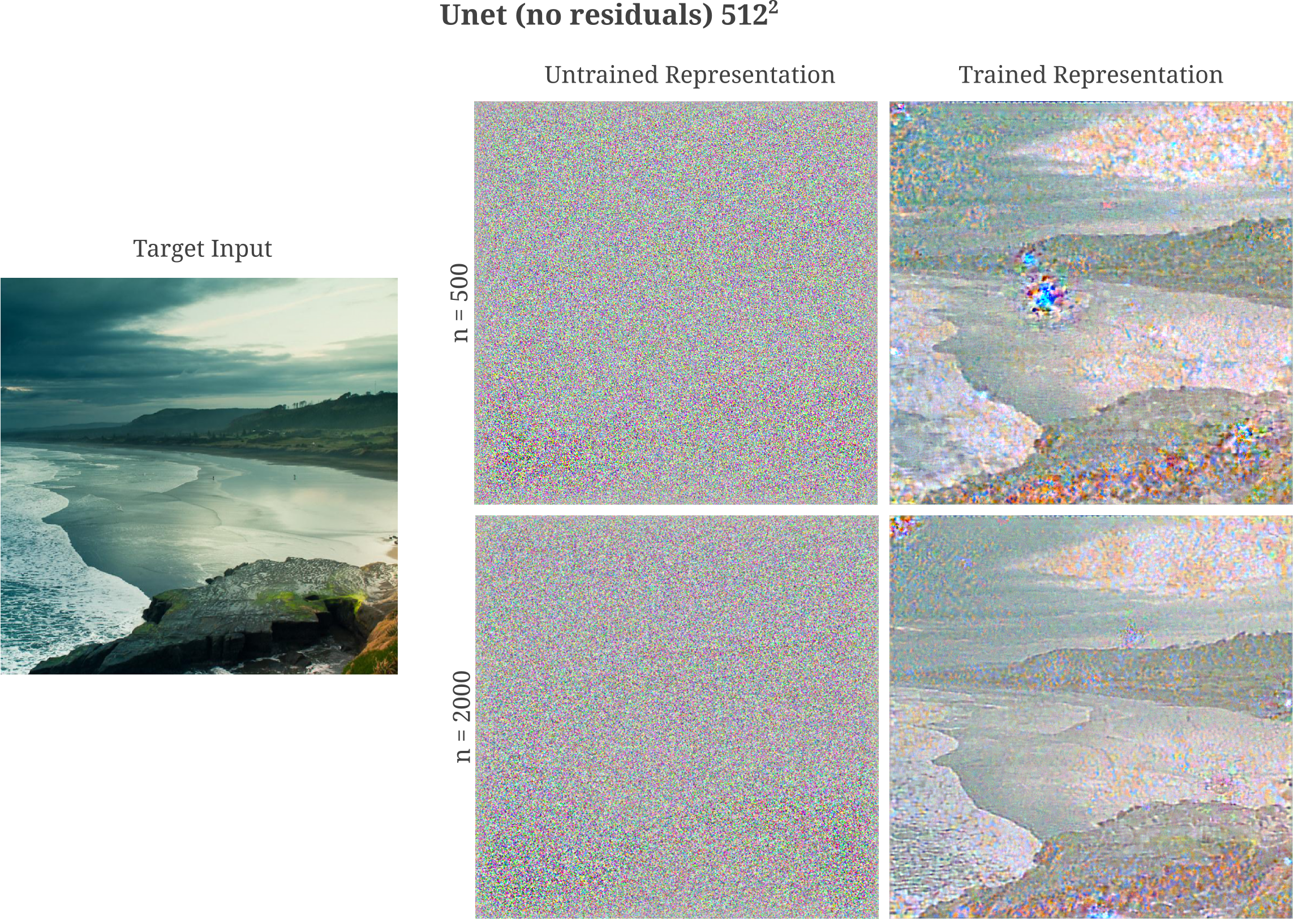The trained model has clearly learned to reduce the noise in the last layer’s representation relative to the untrained model. It may thus be wondered whether autoencoders are capable of learning to de-noise inputs.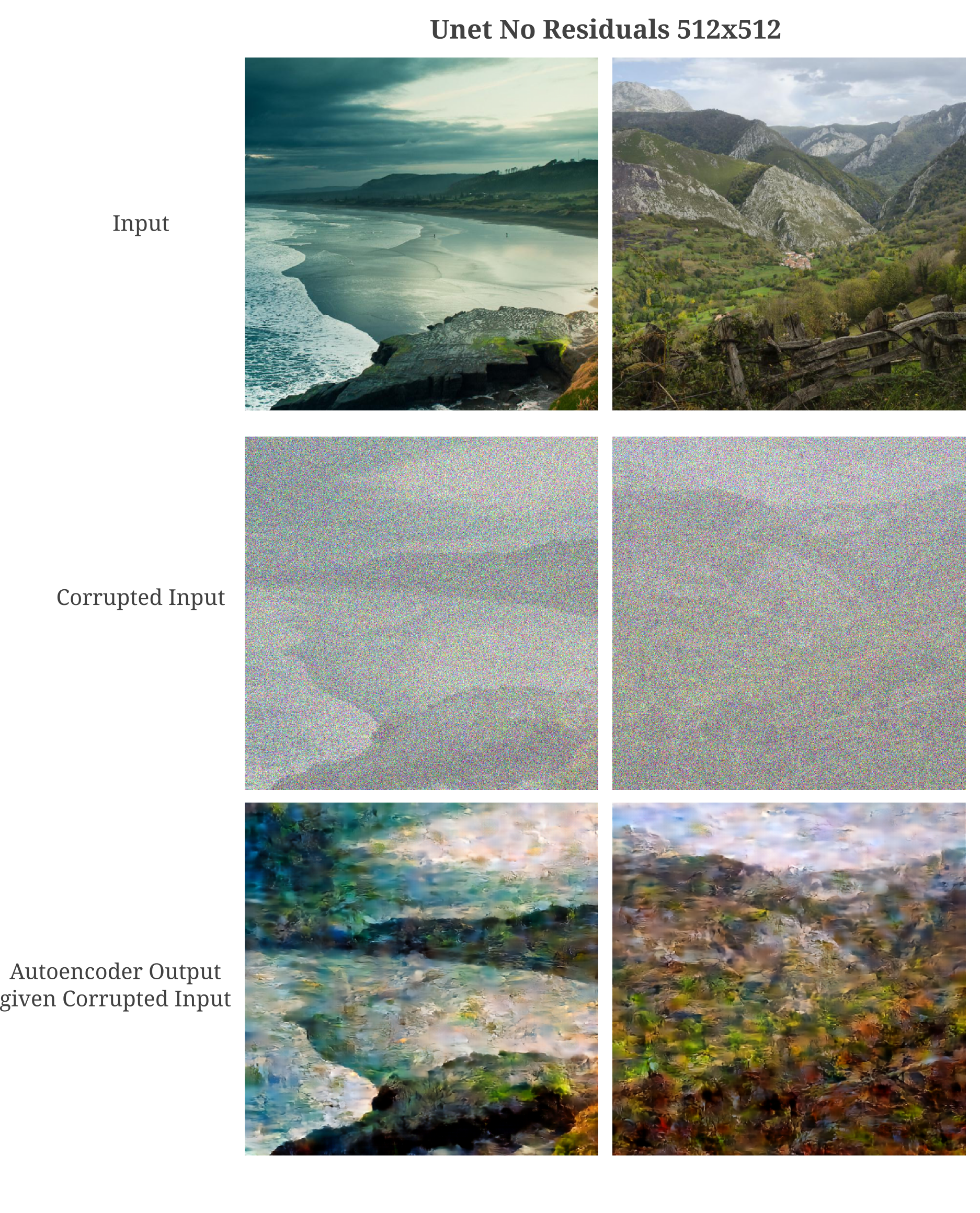The same ability of autoencoders to de-noise is observed for Unet applied as an autoencoder for lower-resolution images, here 64x64 LSUN church images.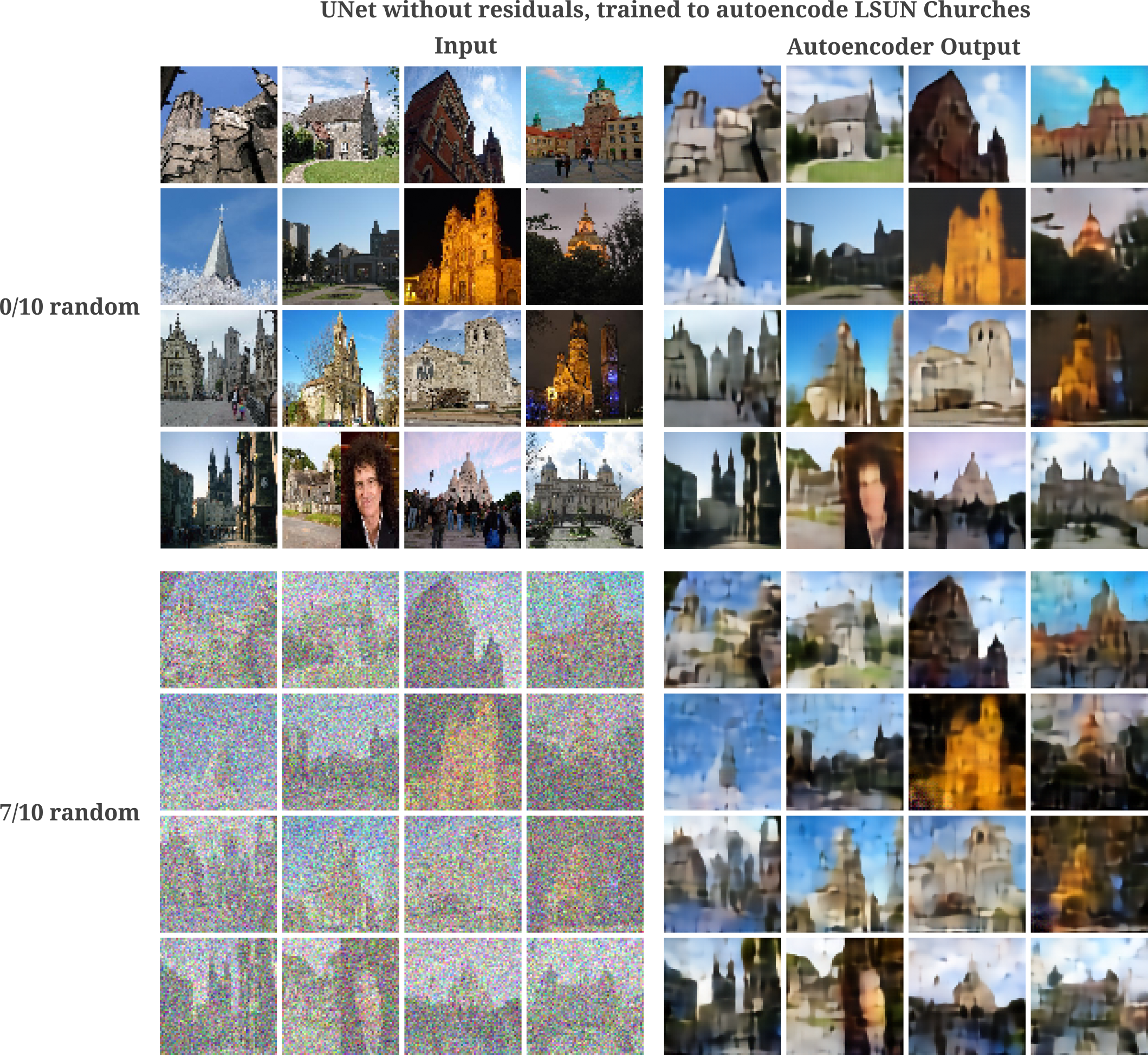With more training, we see that images may be generated even with a large addition of noise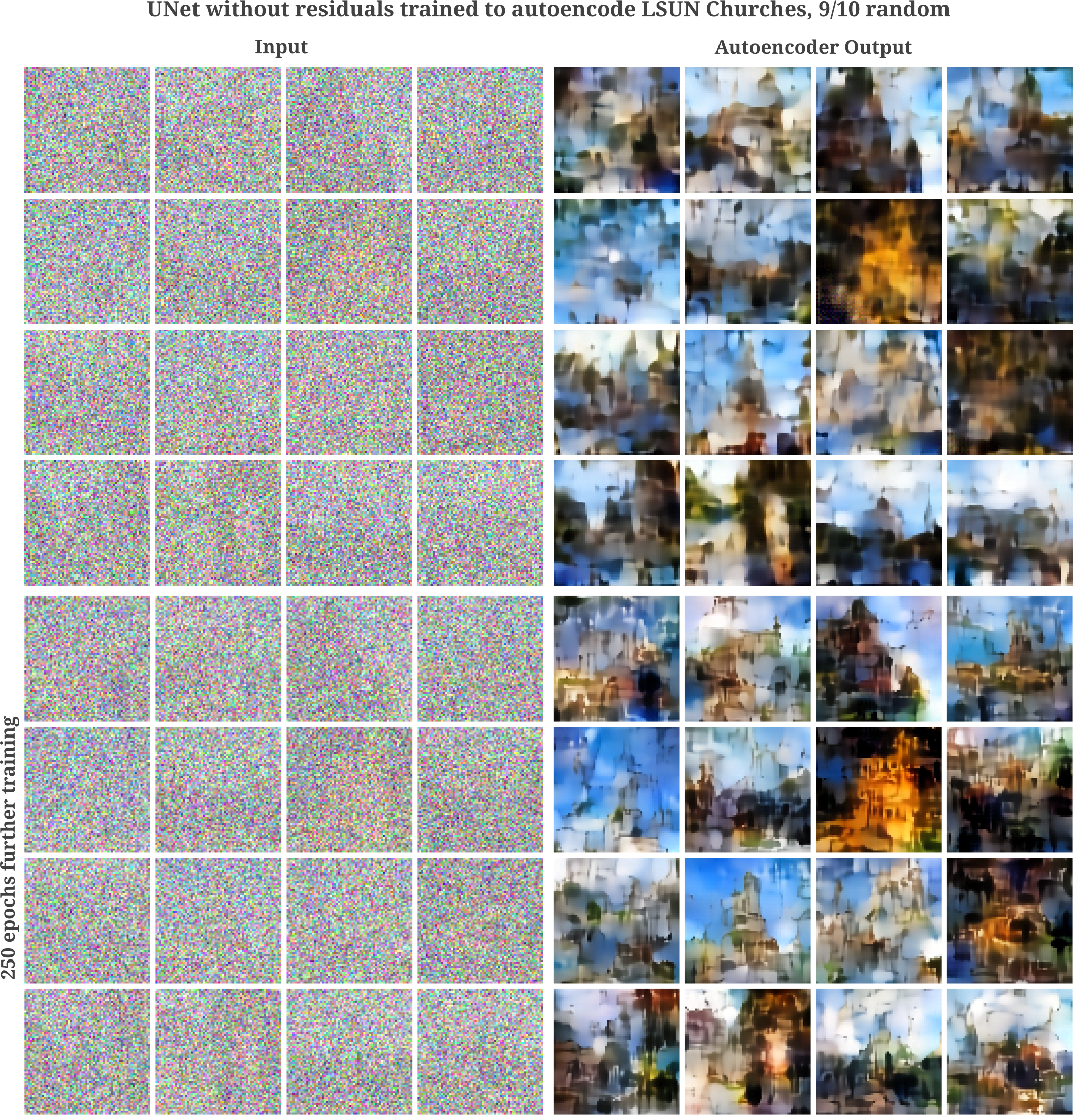and for pure noise, we have the following: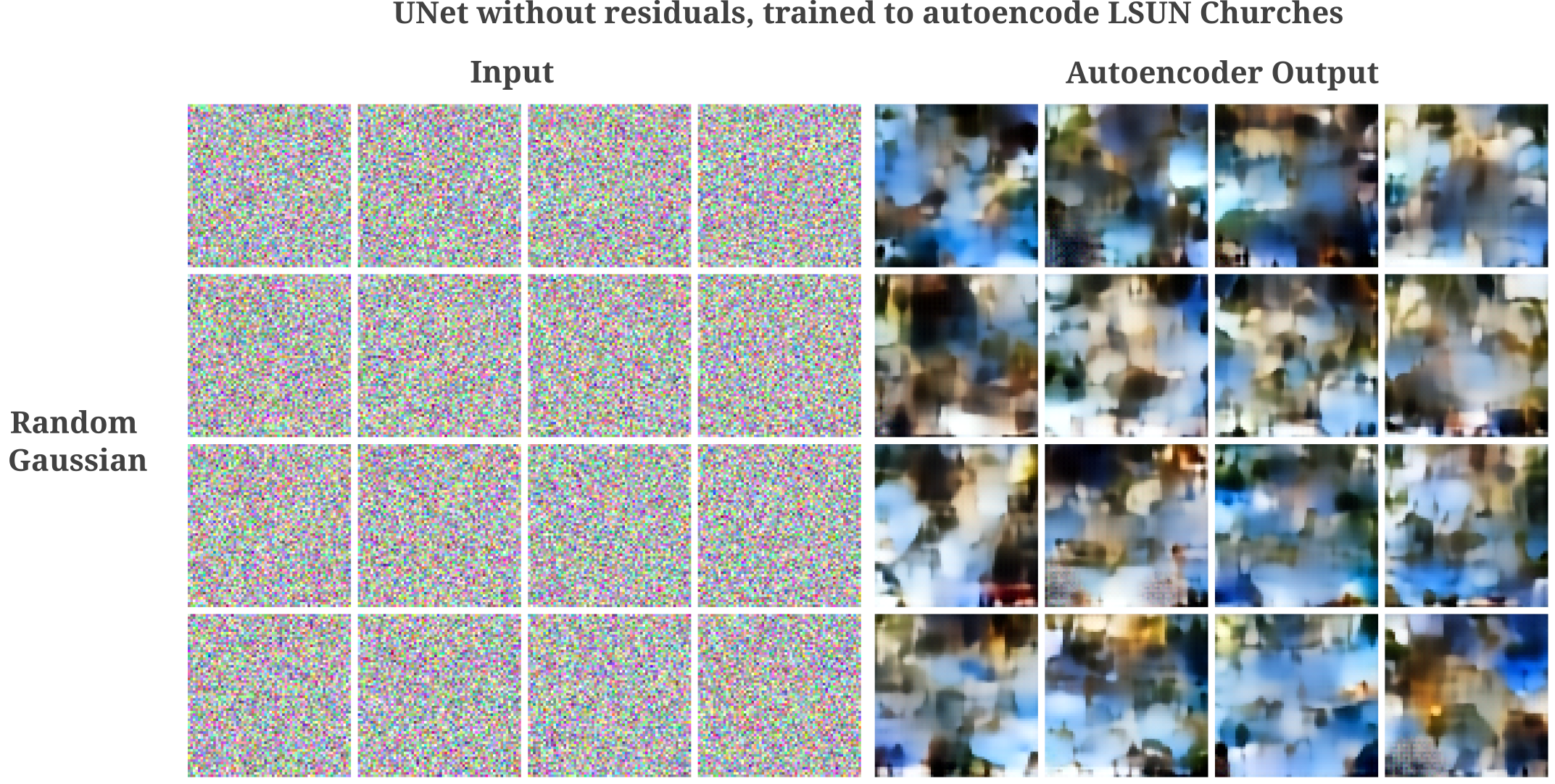Early in this section we have seen that autoencoder training leads to the de-noising of Unet’s representation (in the output layer) of its input. After we have seen how Unet is capable of an extraordinary amount of denoising even without being trained to do so. It may be wondered if these two phenomena are connected, that is, if denoising occurs because the learned representation has less noise than the input. If this were the case then we would expect a noise-corrupted input to lose some of this noise not only in the autoencoder output but also in the autoencoder’s output layer representation of its input. Our expectation is correct, as we can see in the following figure: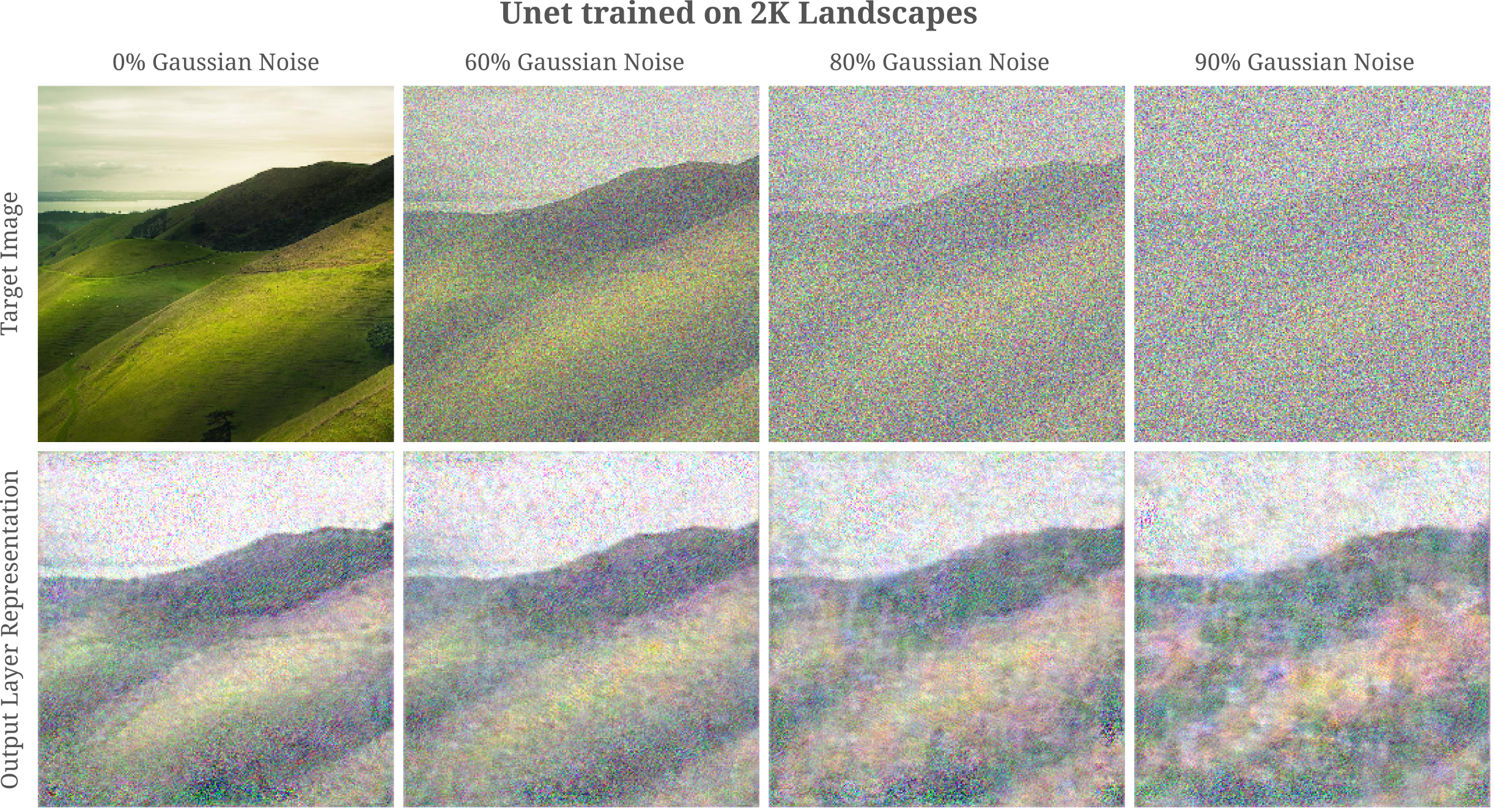It may be wondered then if there is necessarily an equivalence relation between the ability of an autoencoder to accurately represent its input (and hence to de-noise the original representation as we will see in the next section) and the ability to accurately autoencode. The answer here is no, not necessarily: given a powerful enough decoder, most information from the input can be lost and an accurate encoding can be made. For example, if a restrictive three-hidden-layer module is inserted into the Unet (see later sections for more details) then the model is capable of accurate autoencoding (and substantial de-noising) but does not accurately represent its input as shown in the following figure.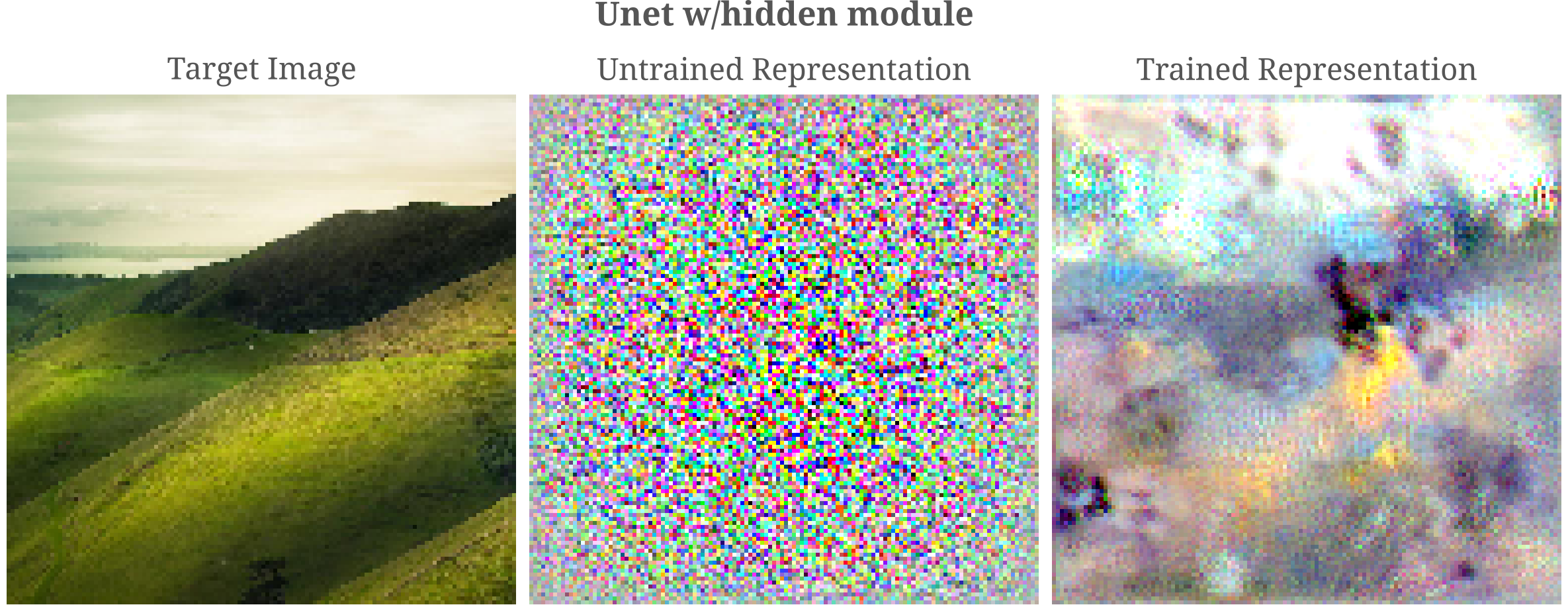### Why Noninvertibility introduces Gaussian Noise in Input Representations

So far we have seen empirically that autoencoders are capable of removing noise from an input even when they are not trained to do so, and rather are tasked with copying the input to the output. This may be understood to be equivalent to the finding that autoencoders learn manifolds that are not noisy, and map arbitrary inputs (even noisy ones) to that manifold.

There is another related phenomenon that may also play a role in the ability of autoencoders to denoise: the non-invertibility of transformations in autoencoders introduces noise into the representations of the input, but much of this noise is removed from the representation during the training process.

Noise is introduced between non-invertible (or approximately non-invertible) transformation representations for the simple reason that many possible inputs may yield one identical output. These many possible inputs resemble Gaussian noise if the transformation in question contains a large number of independent elements, which is indeed the case for typical deep learning models.

To see why non-invertibility for a transformation consisting of a large number of independent elements results in the introduction of Gaussian noise in the output’s representation of the input, first define the transformation of some layer of our model to be a function $f: \Bbb R^n \to \Bbb R^m$ where the input $a$ contains $n$ elements and the output $m$ elements. For a fully connected feedforward deep learning model, $f$ is a composition of $m$ functions $f_1, f_2, … f_m$ each taking all $n$ elements as their inputs as follows:

$f = \{ f_1(a_1, a_2, ..., a_n), f_2(a_1, a_2, ... a_n), ... , f_m(a_1, a_2, ..., a_n)\}$

where each $f_m$ is typically a degree one polynomial of the input,

$f_m(a_1, a_2, ..., a_n) = w_{1, m} a_1 + w_{2, m} a_2 + \cdots + w_{n, m} a_n$

Now consider the significance of the output of $f$ being non-unique with respect to the input: this means that many different inputs $a$ yield some identical $f(a)$. Without prior knowledge besides the given values of $w$ which define the output $y = f(a)$, we can therefore form $m$ probability distributions describing the likelihood of all possible inputs $x_i$ that satisfy $f(x_i) = y$.

Recalling that the output $f_m$ is a polynomial of all elements of $a$, we can express $f_m$ as follows

$f_m(a) = w_{1, m} * p(a_1 | f(a)) + w_{2, m} * p(a_2 | f(a)) + \cdots + w_{n, m} * p(a_n | f(a))$

where $p(a_n \vert f(a))$ signifies the probability of input element $a_n$ given the output $f(a)$. We can draw random variable samples from these probability distributions, $A_i \sim p(a_i \vert f(a))$ such that $f_m(a)$ can be expressed as

$f_m(a) = w_{1, m} * A_1 + w_{2, m} * A_2 + \cdots + w_{n, m} * A_n$

Ignoring for the present approximate non-invertibility, for absolute non-invertibility only each distribution $p(a_n)$ is uniform over all indicies $i$ of valid values of element $n$ in the input, $a_{n, i}$ that satisfy

$\{ a_{n, i} : f_m^{-1}(f(a_n))_i \approx a_{n, i} \}$

so that the corresponding probability distributions are as follows:

$p(a_n | f(a)) = \mathcal{U} (a_{n, i})$

Considering approximate non-invertiblity once again, the distributions $p(a_n \vert f(a))$ are typically not uniform and are indeed difficult to express exactly. The exact nature of each distribution is irrelevant, however, as we can apply the central limit theorem to $f_m(a)$ because it is the sum of many independent variables. The classical central limit theorem states that for independent and identically distributed random variables $X_1, X_2, X_3, …$

$\lim_{n \to \infty} \Bbb P(X_1 + \cdots + X_n \leq n \mu + \sqrt{n} \sigma x) \\ = \int_{-\infty}^{x} \frac{1}{2 \pi}e^{-y/2} dy$

where the expectation value $\Bbb E(X) = n \mu$ and the standard deviation is $\sqrt{n} \sigma$. Therefore for independent and identically distributed random variables $A_1, …, A_n \sim p(a_1 \vert f(a)), …, p(a_n \vert f(a))$ we may safely assume that the distribution $p(f_m(a))$ is Gaussian if the weights $w_{1, m}, …, w_{n, m}$ are identical or near-identical as is usually the case upon model initialization where the weights are sufficiently close to the origin, $\vert w_{i, j} - 0 < \epsilon \vert \; \forall i, j$.

For the problem at hand, we are guaranteed independence when choosing $A_1, …, A_n$ but typically not identical distribution unless certain assumptions are made about the set of possible valid inputs that yield $f(a)$ or on the set of weights $w$. But we can also forego these assumptions if we instead rely on Lindeberg’s central limit theorem, which stipulates only that the random variables in question have finite variance, independence, and satisfy Lindenberg’s condition.

Do these random variables in question satisfy Lindenberg’s condition? The condition is

$\lim_{n \to \infty} \frac{1}{s_n^2} \sum_{k=1}^n \Bbb E \lbrack (X_k - \mu_k)^2 \cdot 1_{|X_k - \mu_k|< \epsilon s_n} \rbrack = 0$

for all $\epsilon > 0$ where

$s_n^2 = \sum_{k=1}^n \sigma^2_k$

which for our dataset is not entirely easy to show. But happily a strict subset of sequences of distriutions $X_1, X_2, …, X_n$ that fulfill Lindenberg’s condition also satisfy Lyapunov’s condition, where defining

$s^2_n = \sum_{i=1}^n \sigma^2_i$

for some $\delta > 0$ there is

$\lim_{n \to \infty} \frac{1}{s^{2 + \delta}_n} \sum_{i=1}^n \Bbb E \left[ |X_i - \mu_i|^{2+\delta} \right] = 0$

The Lyapunov condition requires the sum of the expectations of the difference between random variable $X_i$ and the mean of that random variable $\mu_i$ to grow smaller and smaller than the sum of variances of those distributions as the number of random variables increases. It is straightforward to see that this condition is upheld if all $X_i$ are approximately normal themselves, as many more samples are drawn within one unit of variance of the mean than otherwise for each random variable.

For $\delta = 1$ we can check this condition empirically for a simplified MLP.

This is significant because the central limit theorem states that addition of many independent distributions (of any identity) tends towards a Gaussian distribution. Therefore the addition of any set of distributions of possible values of $p(a_1)$ tends towards the Gausian distribution as $n \to \infty$.

It should be remembered, however, that this is only true if the weights $w_{1, m}, …, w_{n, m}$ on the distributions are approximately identical, and if this is not true then we are by no means guaranteed that the noise will be Gaussian distributed after training. This is because training typically leads to changes in weights such that weights are no longer approximately identical.

One note on the preceeding argument: it may at first seem absurd to suppose that $A_1, A_2, …, A_n$ are independent random variables because if one chooses a certain value for the first element of $a_1 = A_1$ then there is no reason to suppose that this does not limit the possibilities for choosing subsequent values $a_2, …, a_n$. But this does not mean that random variables $A_1, A_2, …, A_n$ are dependent because the choosing of one random variable from $A_n \sim p(a_n \vert f(a))$ does not affect which element is chosen from any other distribution, rather only that the joint conditional distribution $p(a_1, a_2, …, a_n \vert f(a))$ is extremely difficult to explicitly describe.

It may also seem strange to assume that we assume finite variance for $A_1, …, A_n$ being that for an undercomplete linear transformation an infinite number of inputs $a_i$ may identically yield one output $f(a)$. This may be done because the input generation process is effectively bounded (only generated inputs $a_g$ near the starting input $a_0$ will be found via gradient descent), and the same is true of gradient updates during the training process.

### Image Generation with an Autoencoder Manifold Walk

Earlier on this page we have seen that even very large and overcomplete autoencoders do not learn the identity function but instead capture useful features of the distribution of inputs $p(a)$. We were motivated to investigate this question by the earlier findings that deep learning classification models tend to generalize even when they are capable of memorization, and that deep layers are typically incapable of exactly representing the input due to approximate and exact non-invertibility.

We can postulate that the theory behind generalization of classifiers also applies to autoencoders as long as they are trained using gradient descent, as there is no stipulation in the original work that the dimensionality of the output is less than the input, or that the models must be trained as classifiers (ie with one-element $\widehat y$ target values).

Likewise, it seems fairly obvious that an inability of a deep layer (which could be an autoencoder output) to represent the input at the start of training would also prevent memorization to some extent, being that it would be highly unlikely for a model to learn the identity function if deeper layers cannot ‘know’ exactly what the input looks like.

We next saw how autoencoders are effective de-noisers, and that at the start of training typical deep learning archictures induce Gaussian noise in subsequent layers such that the model must learn to reduce such internal noise during training. Being that there is no intrinsic difference between internal and input-supplied Gaussian noise, it is not particularly surprising that sufficiently deep autoencoders are capable of powerful noise reduction.

This being the case, it should be possible to generate inputs from the learned distribution $A \sim \widehat{p}(a)$ assuming that the learned distribution approximates the ‘true’ data distribution, ie $\widehat{p}(a) \approx p(a)$.

For overcomplete autoencoders, however, we are presented with a challenge: how do we navigate the latent space to synthesize a sample, given that for a high-dimensional latent space may be large such that only a small subregion of this space is actually explored by the model’s samples? For example, say the dimensionality of the smallest hidden layer of the autoencoder (which we take to be the latent space) is larger than the dimensionality of the input. Simply assigning the values of this latent space as a random normal distribution before forward propegating this information to the output is not likely to yield an image representative of $p(a)$ unless samples of $p(a)$ yielded a similar distribution in that latent space.

This is extremely unlikely unless some constraints are placed on the model, and indeed a very similar model to the one we have considered here has been investigated. These models are called variational autoencoders, and although effective in many aspects of probability distribution modeling they suffer from an inability to synthesize sharp images $A$, which can be attributed to the restrictive nature of mandating that the latent space be normally distributed.

Instead of enforcing restrictions on our model, we can instead map inputs to the learned manifold and perform a walk on this manifold, effectively creating a Markov process to generate images. Suppose we split the Unet autoencoder into two parts, an encoder $\mathcal E$ that takes inputs $a$ and yields a hidden space vector $h$ along with a decoder $\mathcal D$ that takes as an argument the hidden space vector $h$ and yields the synthesized image $g$

$g = \mathcal D(\mathcal E(a)) = \mathcal D(h)$

Being that $h$ is not low-dimensional but $\mathcal D(\mathcal E(a))$ has learned $p(a)$, we can first find the hidden space vector $h$ corresponding to some set of $A \sim p(a)$

$h_1' = \mathcal E(a)$

Adding a small amount of normally distributed noise to this latent space gives a new latent space point

$h_1 = h_1' + \mathcal N(h_1'; 0, \epsilon)$

Then using this latent space vector the corresponding synthesized images are

$g_1 = \mathcal D(h_1)$

Now $g_1$ is likely to be extremely similar or identical to $a$ as long as the learned manifold is not too unstable. But if we then repeat this procedure many times such that the Markov chain may be expressed as

$h_{n+1}' = \mathcal E(g_n) \\ h_{n+1} = h_{n+1}' + \mathcal N(h_n'; 0, \epsilon) \\ g_{n+1} = \mathcal D(h_{n+1})$

we find that the latent space is effectively explored. For a Unet trained on 256 $512^2$ resolution images of landscapes, we have the following over 300 steps on a manifold walk:

Training a generative model on such a small number of images is not likely to yield very realistic inputs, and it appears that the images generated during the manifold walk are locally realistic but globally somewhat incoherent (observe how portions of sky and land tend to pop up over the entire image).

Increasing the dataset size from 256 to 2048 (and decreasing the resolution to $256^2$) we have much more coherent generated images. It is also interesting to note that we see movement between images learned from the training dataset, which is not surprising given that these are expected to exit on the learned manifold.

It is more curious that we see a qualitatively similar manifold even without the addition of noise at each step of the Markov process. More precisely, if we perform the manifold walk as follows

$h_{n+1} = \mathcal E(g_n) \\ g_{n+1} = \mathcal D(h_{n+1})$

we find

This shows us that much of the tendancy of repeated mapping to a manifold to lead to movement along that manifold occurs in party due to that manifold’s instability. We can pfind further evidence that most of the manifold walking ability is due to the manifold’s instability by performing the same noise-less Markov procedure on autoencoders after varying amounts of training. With increased training, the proportion of inputs that walk when repeatedly mapped to a manifold decreases substantially: with 200 further epochs, we find that this proportion lowers from 7/8 to around 1/8.

### Diffusion with no Diffusion

Given that autoencoders are effective de-noisers, we can try to generate inputs using a procedure somewhat similar to that recently applied for diffusion inversion. The theory here is as follows: an autoencoder can remove most noise from an input, but cannot generate a realistic input from pure noise. But if we repeatedly de-noise an input that is also repeatedly corrupted, an autoencoder may be capable of approximating a realistic image.

To begin, we use a random Gassian distribution in the shape of our desired input $a_g$

$a_0 = \mathcal N(a; \mu, \sigma)$

where $\sigma$ and $\mu$ are chosen somewhat arbitrarily to be $7/10, 2/10$ respectively. Now we repeatedly de-noise using our autoencoder but on a schedule: as the number of iterations increases to the final iteration $N$, the constant $c$ increases whereas the constant $d$ decreases commensurately.

$a_{n+1} = c * \mathcal D( \mathcal E(a)) + d * \mathcal N(a; \mu, \sigma)$

Arguably the simplest schedule is a linear one in which $c = n / N$ and $d = 1 - (n/N)$. This works fairly well, and for 30 steps we have the following for a Unet trained on LSUN churches (note that each time point $t=n$ corresponds to the model’s denoised output rather than $a_n$ which denotes a denoised output plus a new noise).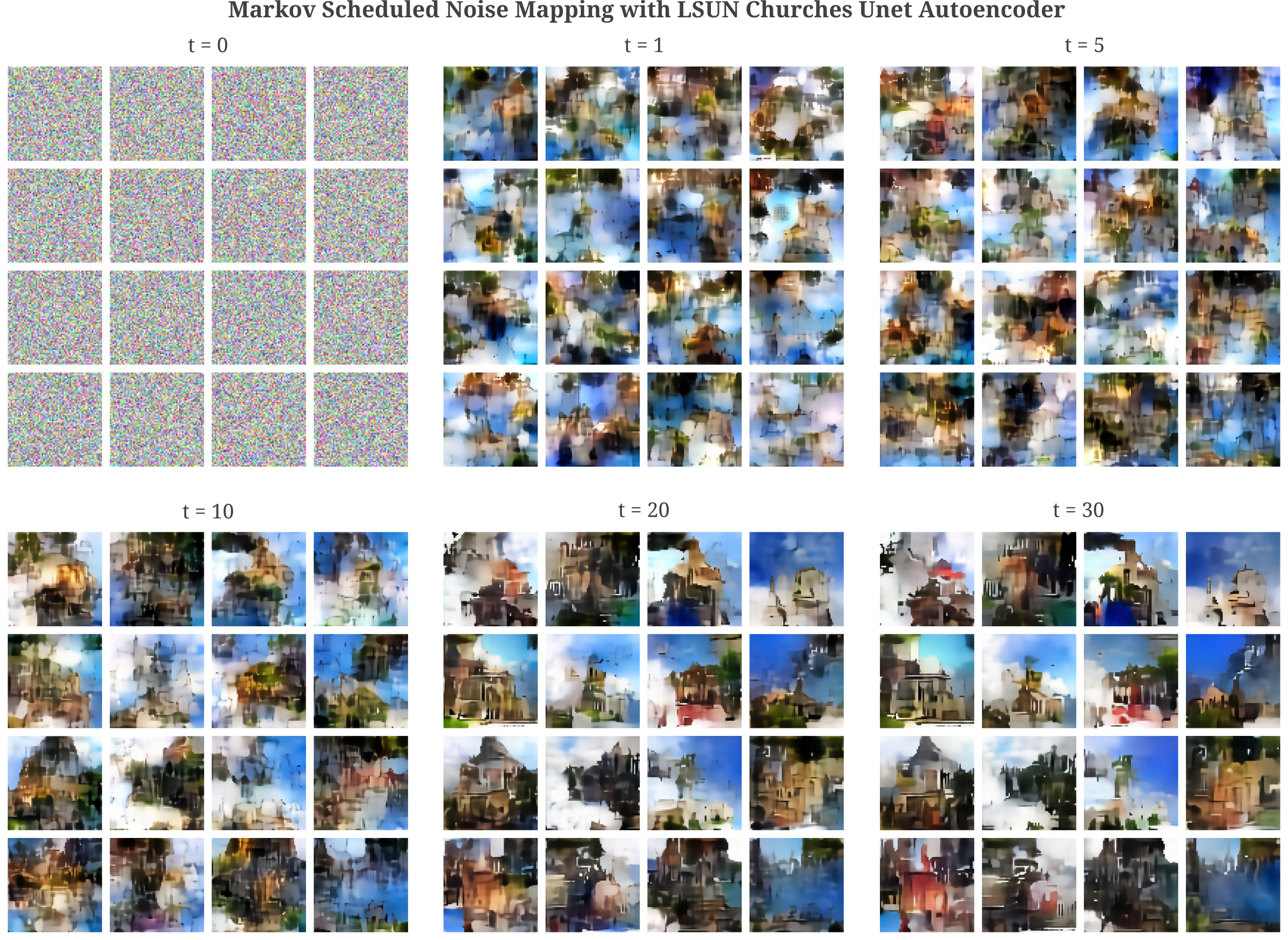And for the same model applied to generate images over 200 steps, we have

This method of continually de-noising an image is conceptually similar to the method by which a random walk is taken around a learned manifold, detailed in the last section on this page. Close observation of the images made above reveal very similar statistical characteristics to those generated using the random manifold self-map walk in the video below

It is interesting to note that these statistical characteristics (for example, the dark maze-like lines and red surfaces on buildings that make a somewhat Banksy-style of generated image) are specific to the manifold learned. Observe that a similar model, Unet with a hidden MLP, as follows: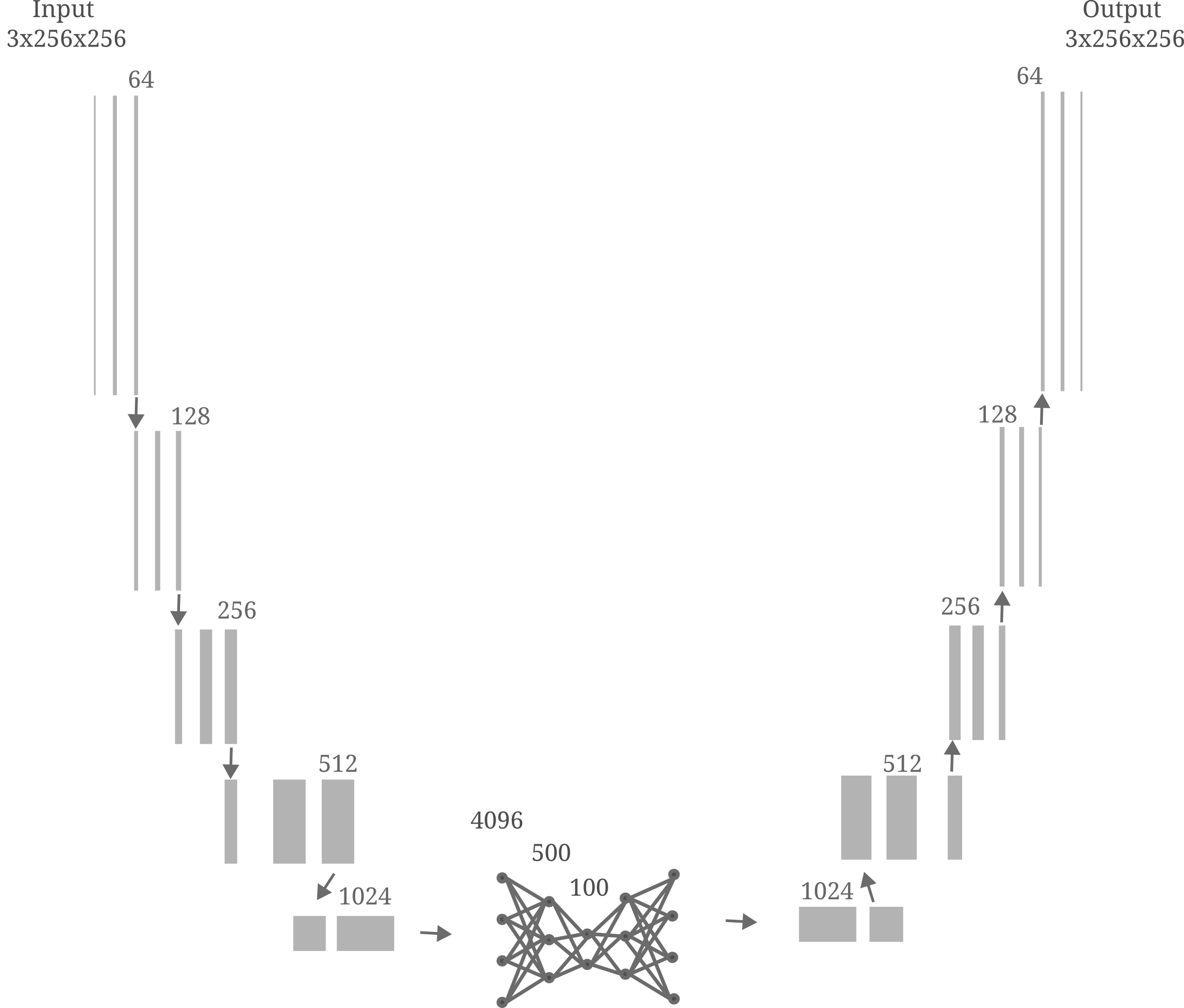is capable of remarkable de-noising ability but tends to make oil painting-style characteristic changes during the denoising process.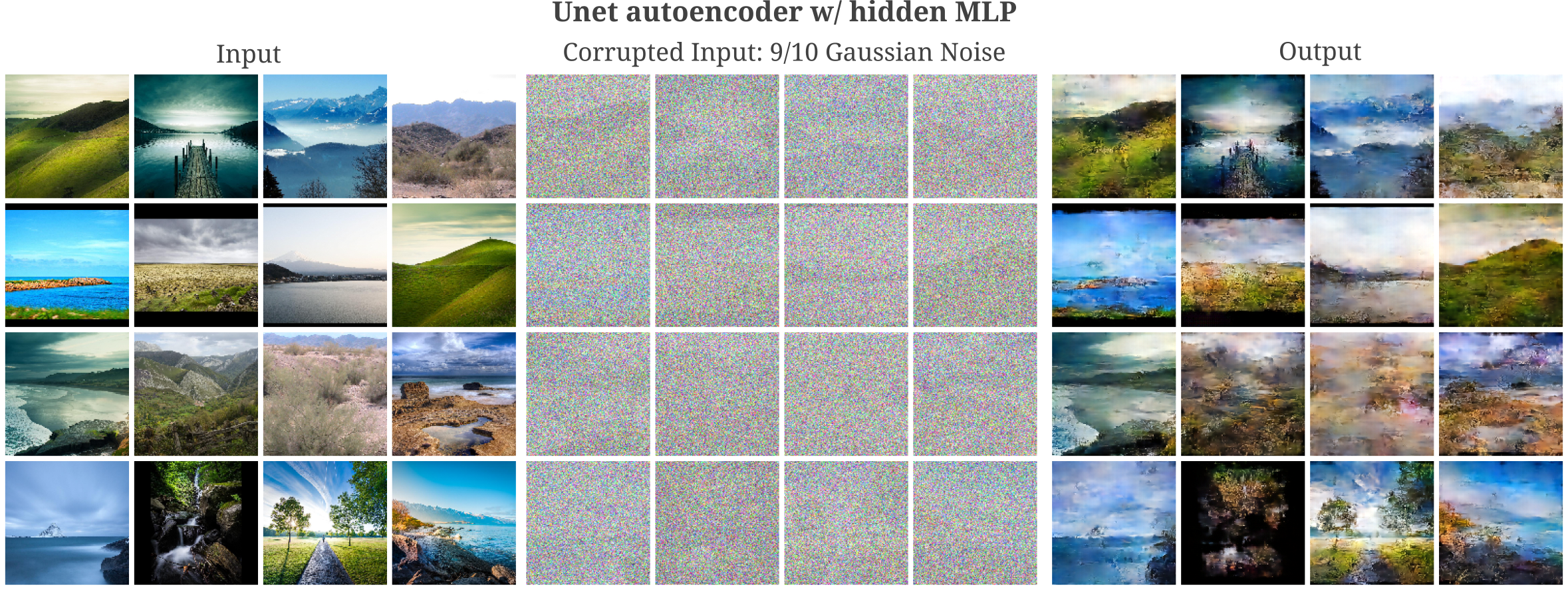This suggests that the manifold learned when autoencoding images of landscapes (by this particular model) will also have oil painting-like characteristics. Indeed this appears to be the case, as when we use the diffusion-like markov sampling process to generate input images, we find that most have these same characteristics as well.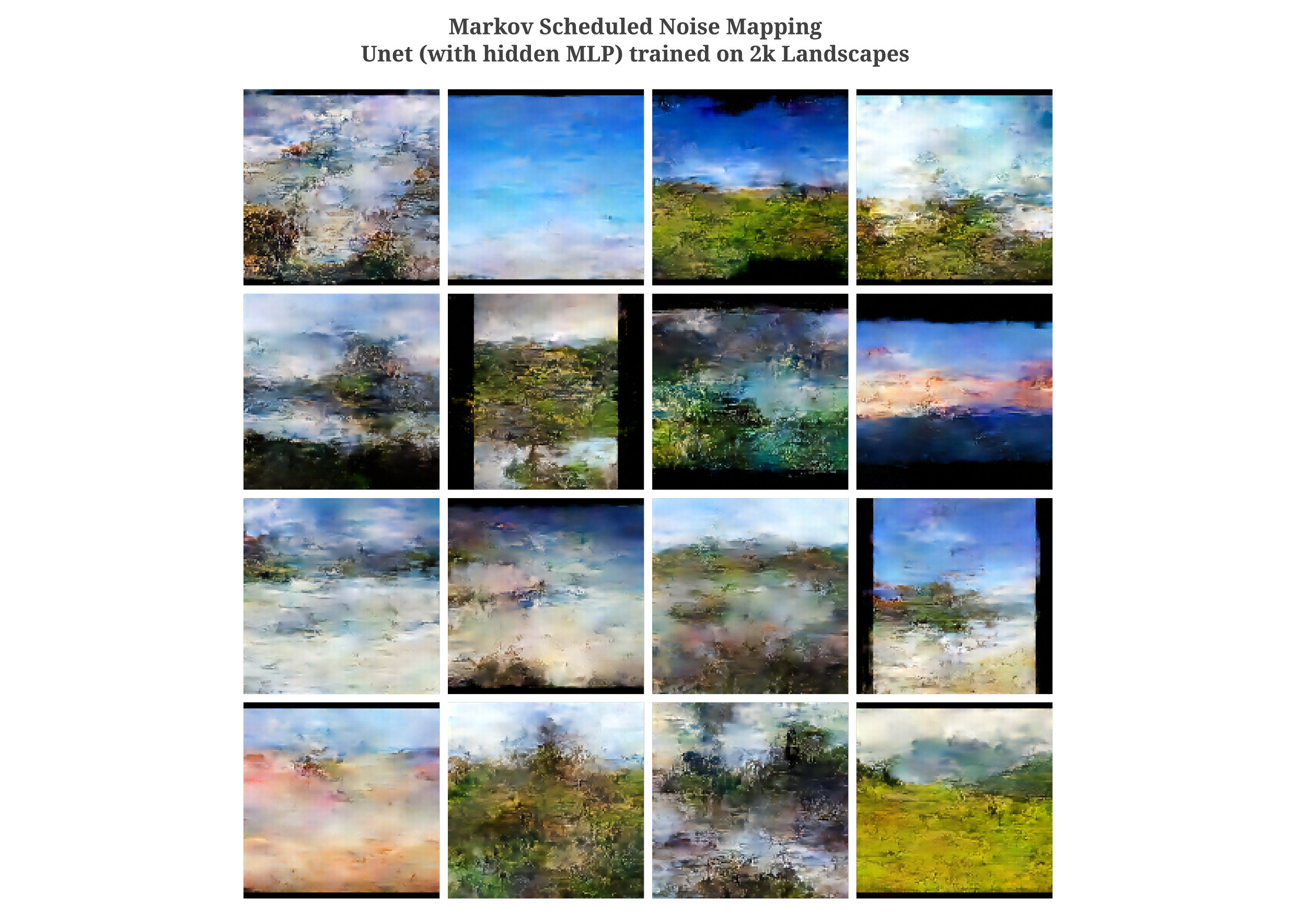Increasing the number of iterations of the markov sampling process to 200, we have

For an increase in sampled image detail, one can increase the number of feature maps per convolutional layer in the Unet autoencoder architecture. With a model that has twice the layers per convolution as the standard Unet, we have the following: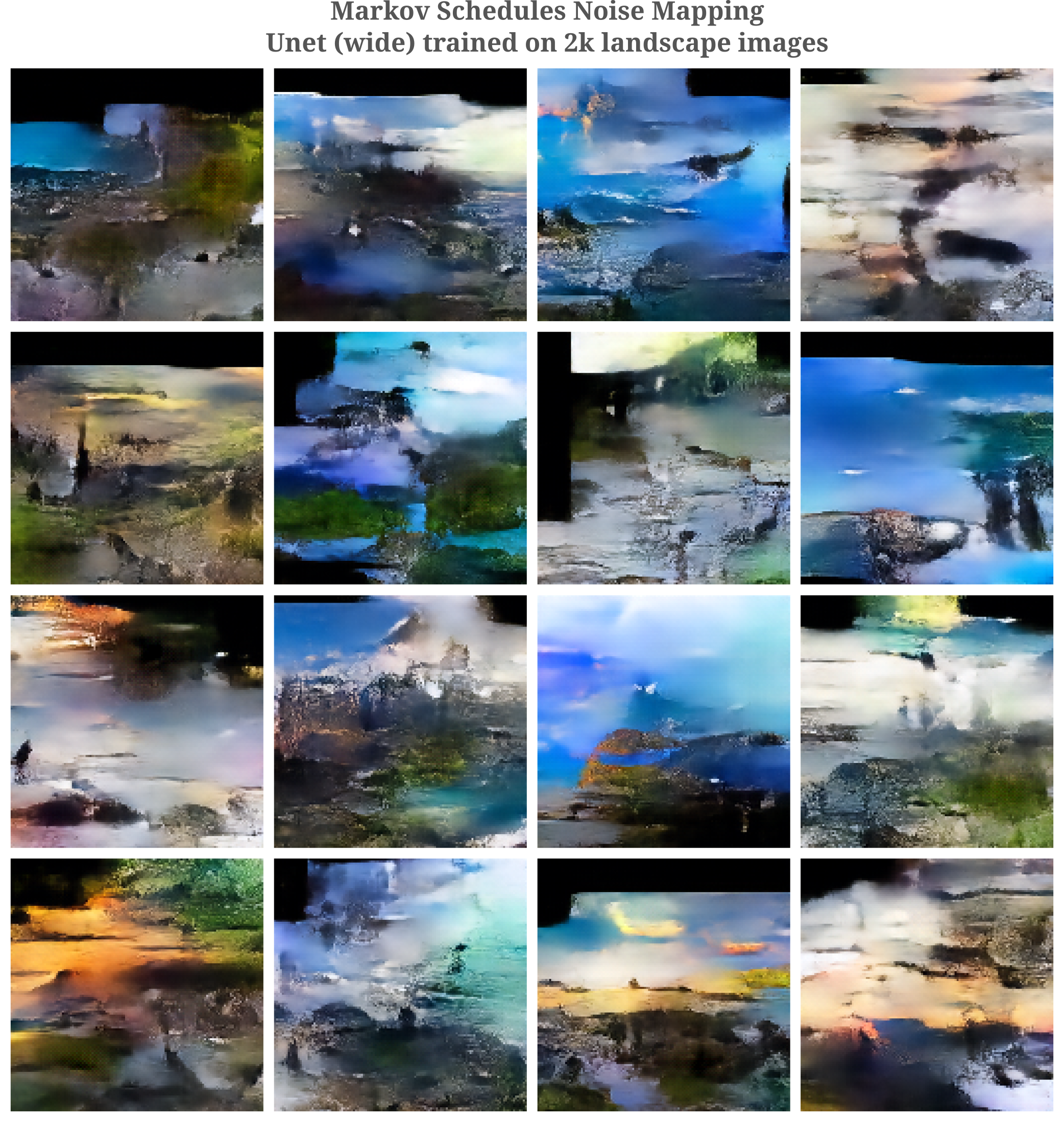Although this model synthesizes images of greater detail and clarity that the narrower Unet, there are signs of greater global disorganization (black borders are often not straight lines, sky sometimes is generated under land).

One way to prevent this disorganization is to increase the number of convolutional layers present in the model (assuming fixed kernal dimension), and another is to employ at least one spatially unrestricted layer such as the fully connected layers above, or self-attention layers. We will investigate self-attention in later sections on this page, and for now will focus on increasing the number of convolutional layers.

To gain intuition for why this is, consider the following example: suppose one were to first take a single convolutional layer as the encoder and single up-convolution as the decoder. There would typically be no model parameters influencing both the pixels in the upper right and lower left of an input assuming that the convolutional kernal is significantly smaller that the input image dimension (as is usually the case). But then there is no guarantee that the model should be able to generate an image observing the ‘correct’ relationship between these two pixels as determined by the training dataset.

On the other hand, consider the extreme case in which many convolutions compose the input such that each image is mapped to a single latent space element, and subsequently many up-convolutions are performed to generate an output. There are necessarily model parameters that control the relationship of every pixel to every other pixel in this case.

Some experimentation justifies this intuition: when we add an extra layer to both encoder and decoder of the wide Unet, we obtain much more coherent images.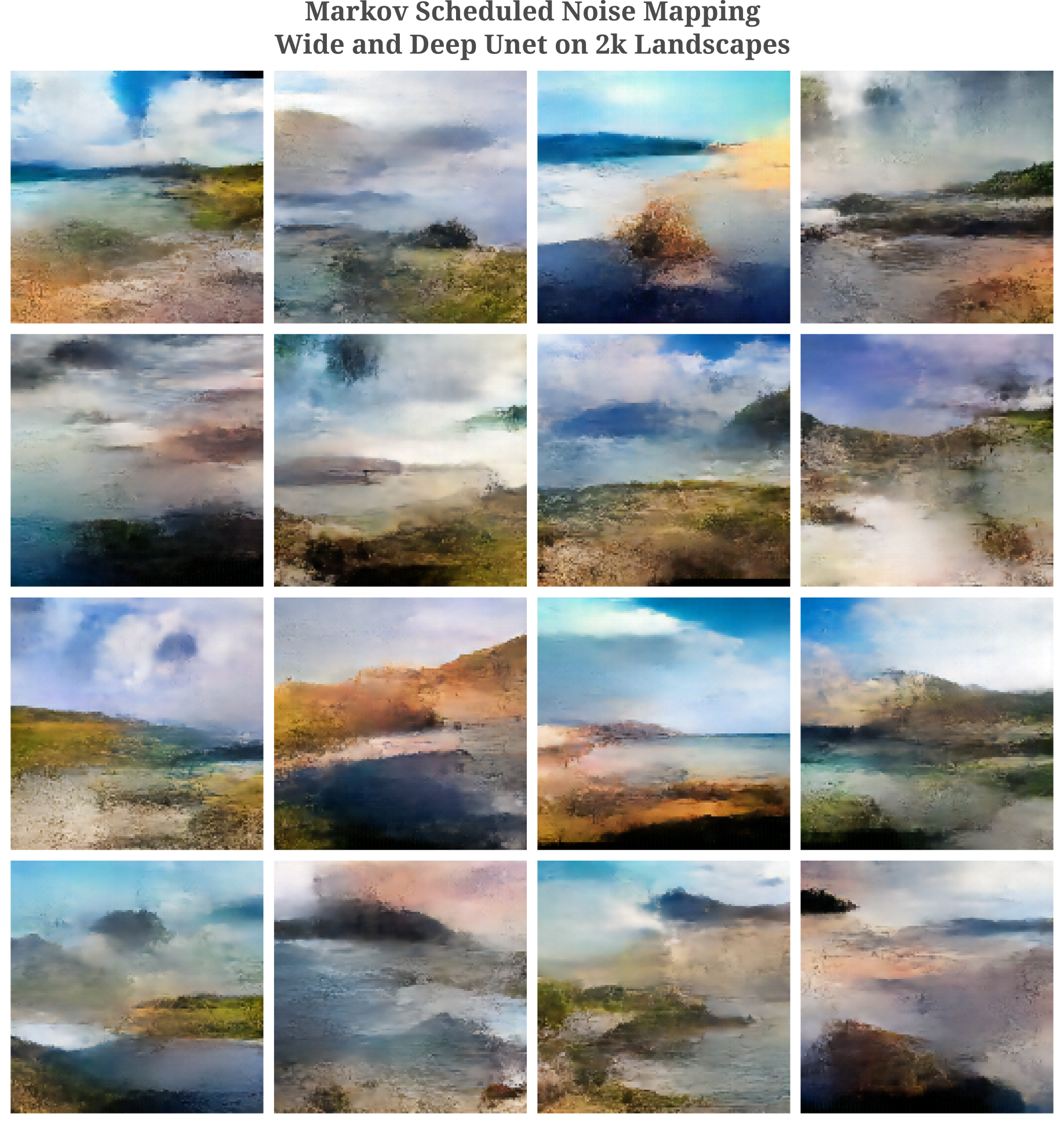It is interesting to note that the extra width (more precisely doubling the number of feature maps per convolutional layer) reduces the number of painting-like artefacts present in the synthesized images after the diffusion-like generation process.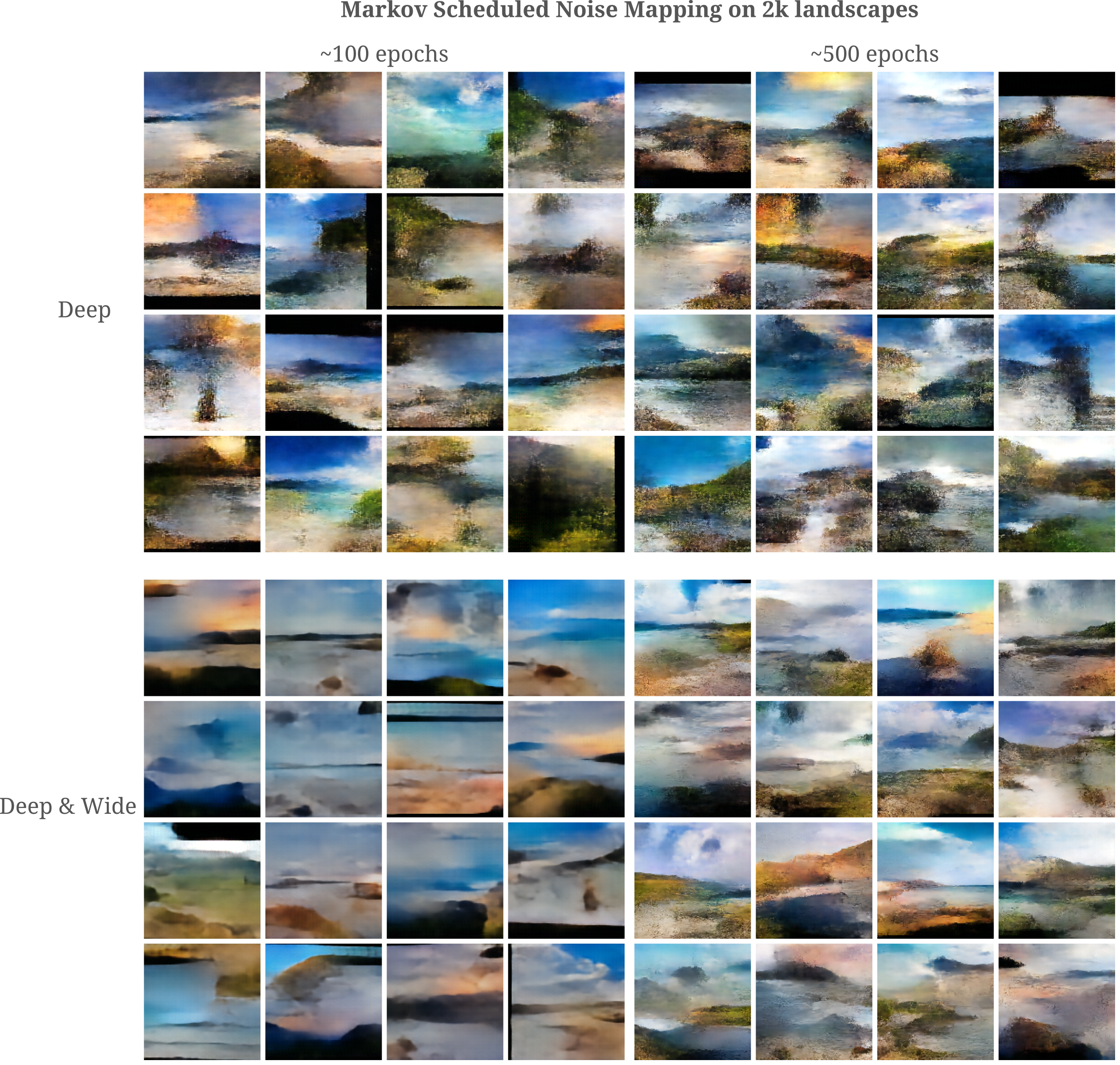### The Role of Batch Normalization in Denoising

Comparing the fully connected autoencoder to the Unet version, it is apparent that the latter is a much more capable denoiser than the former. It is interesting to observe that batch normalization plays a key role in this denoising ability: removing this transformation from all convolutions in Unet results in autoencoder outputs that do not have noise, but are also statistically quite different than the original image. Note that batch norm on this page is not switched to evaluation mode during image synthesis such that the model continues to take as argument the mean and standard deviation statistics of the synthesized images.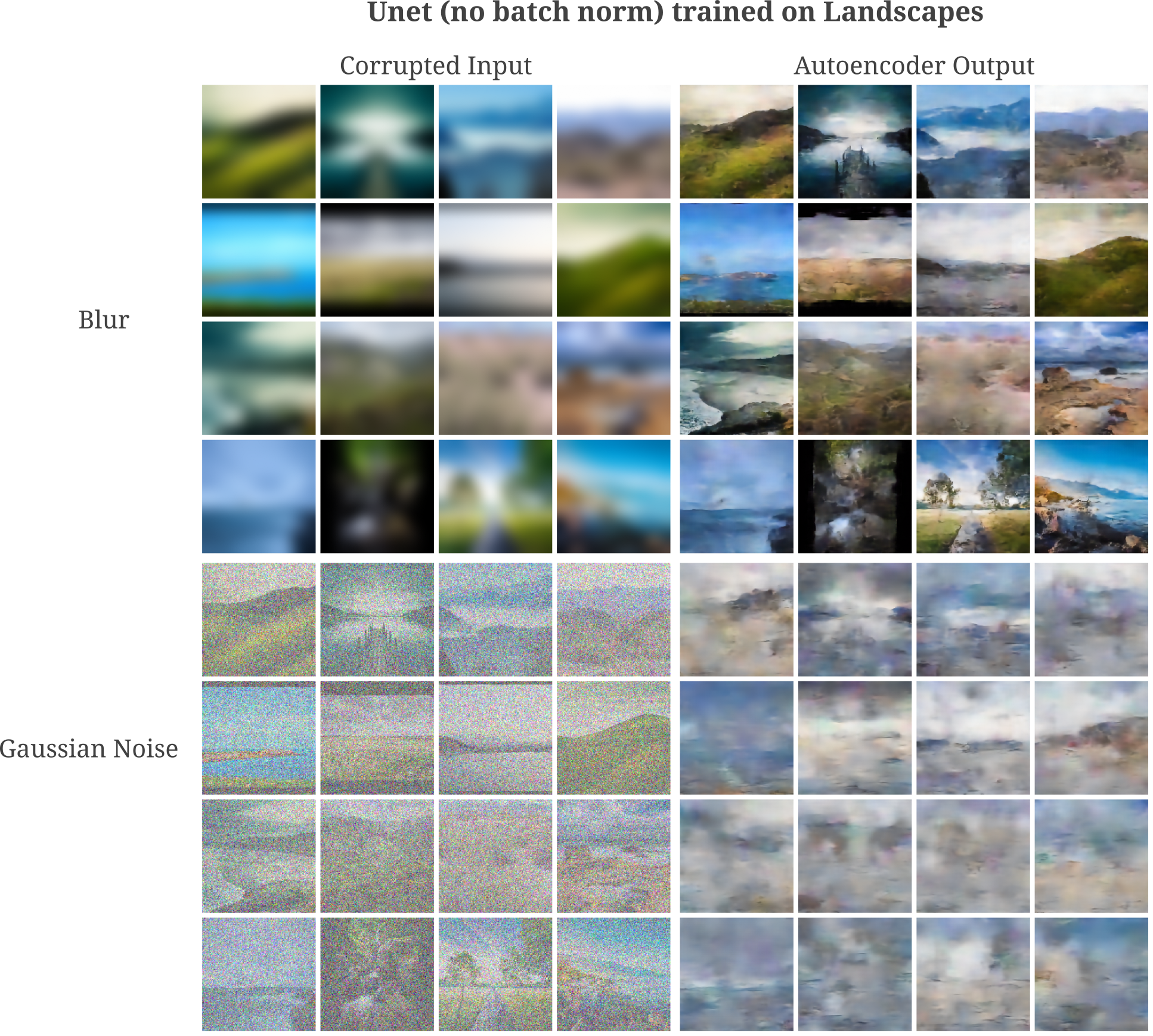We can think of batch normalization as enforcing general statistical principles on the synthesized images. For each layer (also called a feature map) of a convolution, batch normalization learns the appropriate mean and standard deviation accross all samples in that batch necessary for copying a batch of images. During the image synthesis process, batch normalization is apparently capable of enforcing similar statistics on the generated images.

It may be wondered whether batch normalization is necessary for accurate input representation in deep layers of the Unet autoencoder after training. The answer to this question is no, as with or without batch normalization the Unet output has more or less equivalent representation ability of the input after training.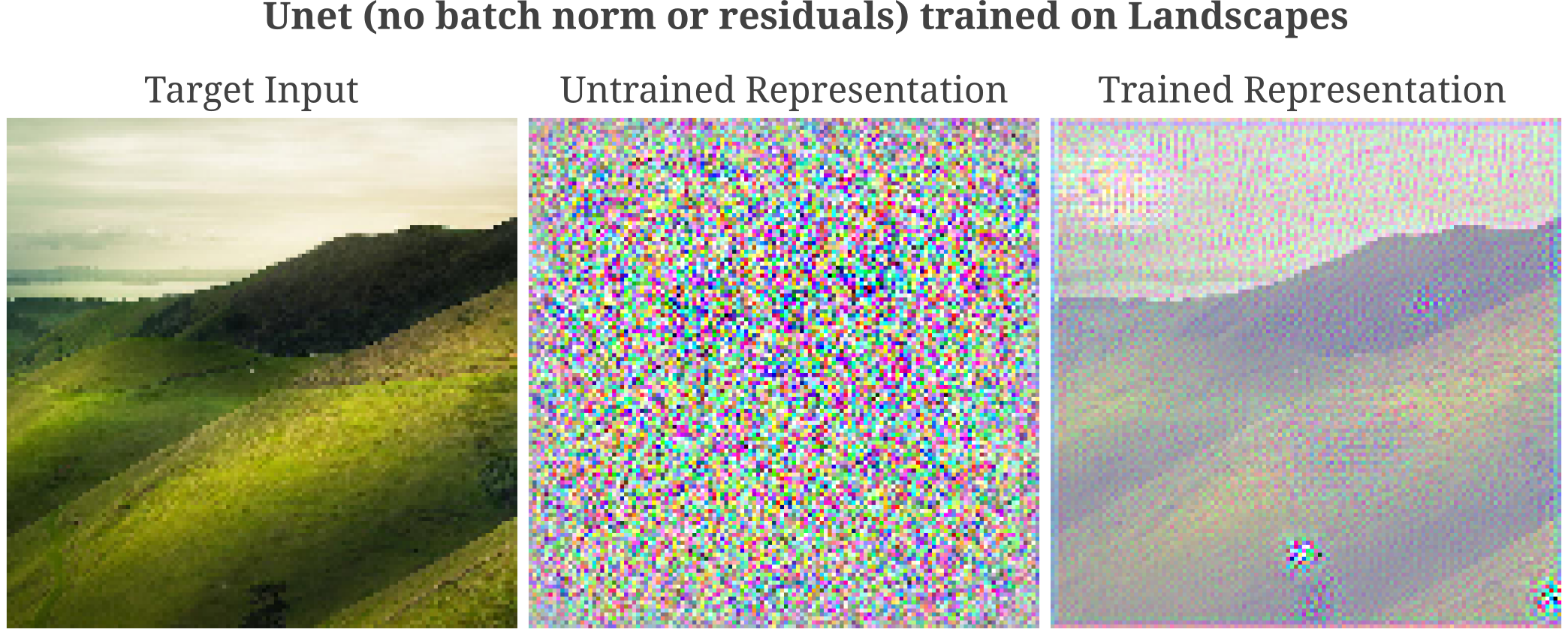### Representations in Unet generative models with Attention

It may be wondered why representation clarity (or lack thereof) would be significant. So far we have seen that the more accurate (ie Vit Large 16) vision transforms contain representations in their hidden layers that mirror those of convolutional models such as ResNet50, in which a near-perfect autoencoding of the input may be made without prior knowledge in early layers but a substantial amount of information is lost during the forward pass, leading to poor input representation accuracy in later layers.

For tasks of classification, the importance of accurate input representation is unclear. One might assume that a poor representation accuracy in deep layers prevents overfitting, but as observed here the gradient descent process itself serves to prevent overfitting regardless of representational accuracy for classifiers. For generative models, however, there is a theoretical basis as to why imperfect deep representation is favorable.

To see why perfect input representation in the output may be detrimental to generative models, take a simple autoencoder designed to copy the input to the output. For a useful autoencoder we need the model $\theta$ to learn parameters that capture some important invariants in the data distribution $p(x)$ of interest. But with perfect input representation capability in the output the model may achieve arbitrarily low cost function value by learning to simply copy the input to output.

Next consider the case of a denoising autoencoder. This model is tasked with recoving the input $x$ drawn from the distribution $p(x)$ followed by a corruption process $C(x) = \tilde{x}$, minimizing the likelihood that the model output $O(\tilde{x}, \theta)$ was not drawn from $p(x)$. Under some assumptions this is equivalent to minimizing the mean squared error

$L = || O(\tilde{x}, \theta) - x ||_2^2$

Now consider the effect of a perfect representation of the input $\tilde{x}$ in the output with respect to minimizing $L$. Then we have

$L = || \tilde{x} - x ||_2^2$

which is the MSE distance taken by the corruption process. Therefore the model is capable of inferring the corruption process without training.

These ideas have practical influence on the design of autoencoders when one attempts to add self-attention modules to the Unet backbone. Attention-augmented Unet architectures are typically used for Diffusion models in order to increase the expressivity of the model in question, and consist of Linear attention between high-dimensional (introduced by Katharopoulos and colleagues) and standard dot-product attention between lower-dimensional convolutional maps in parallel to residual connections.

The architecture we will use employs only linear attention, and may be visualized as follows: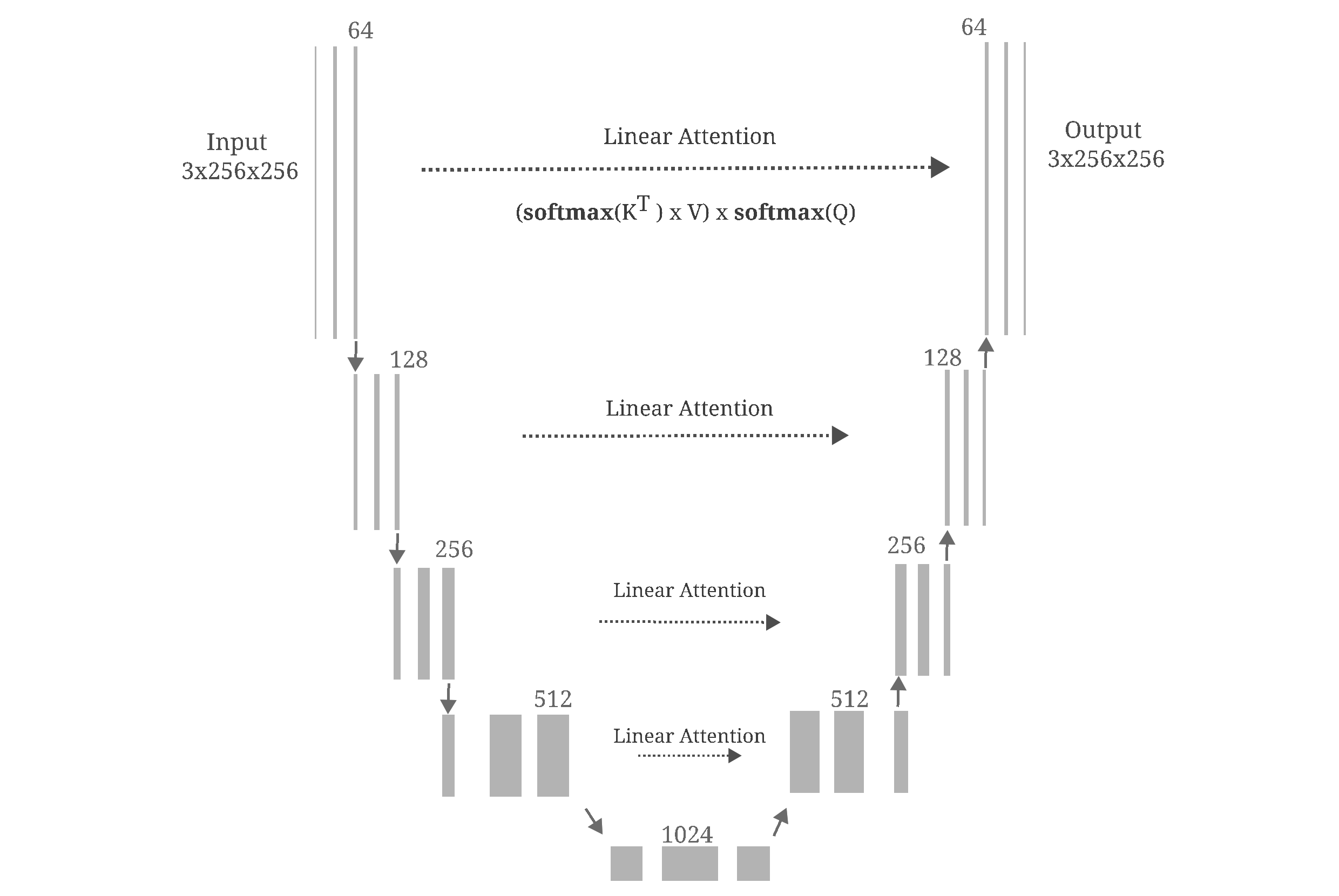But after training, it is apparent that this model is not very effective at de-noising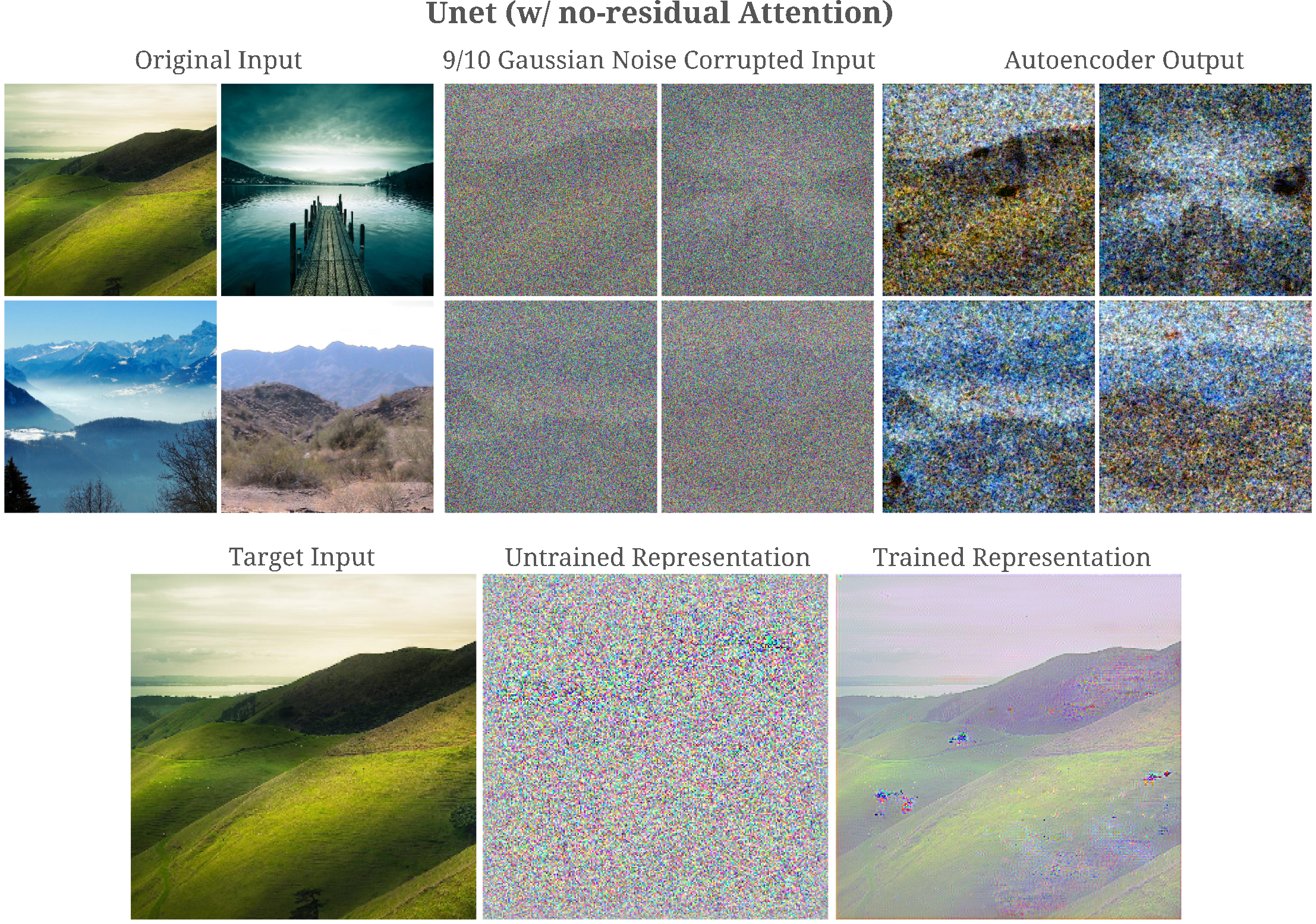It may be wondered how much information is capable of passing though each linear attention transformation. If we restrict our model to just one linear attention transformation and input and output convolutions (each with 64 feature maps), we find that the model tends to learn to copy the input such that the model denoises the input poorly and is capable of near-perfect representation of its input, shown as follows: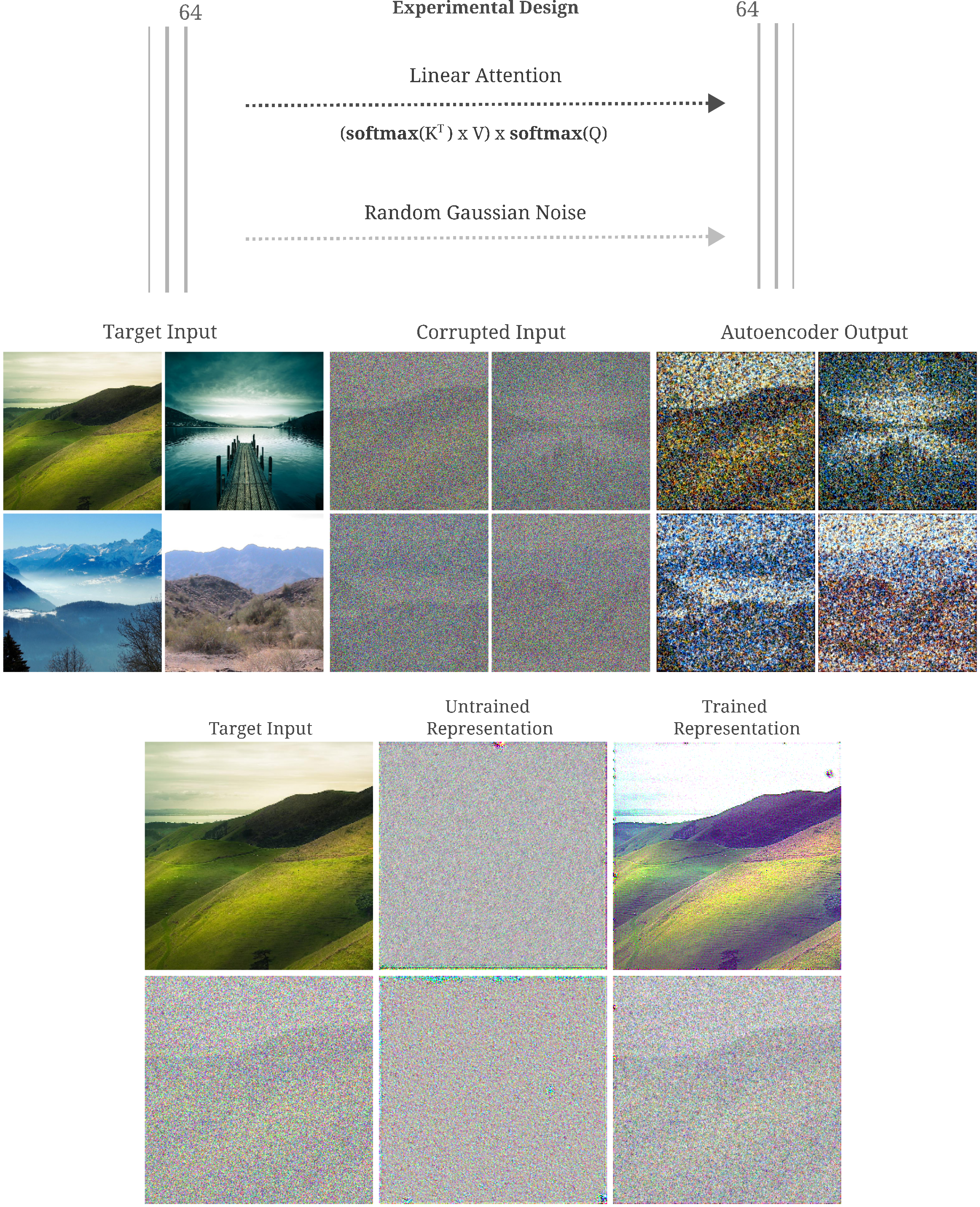Linear attention was introduced primarily to reduce the quadratic time and memory complexity inherent in the dot product operation in the normal attention module with linear-time complexity operations (aggregation and matrix multiplication). If we replace the linear attention module used above with the standard dot-product attention but limiting the input resolution to $32^2$ to prevent memory blow-up (while maintaining the input and output convolutions), we find that after training the autoencoder is capable of a less-accurate representation of the input than that which was obtained via linear attention.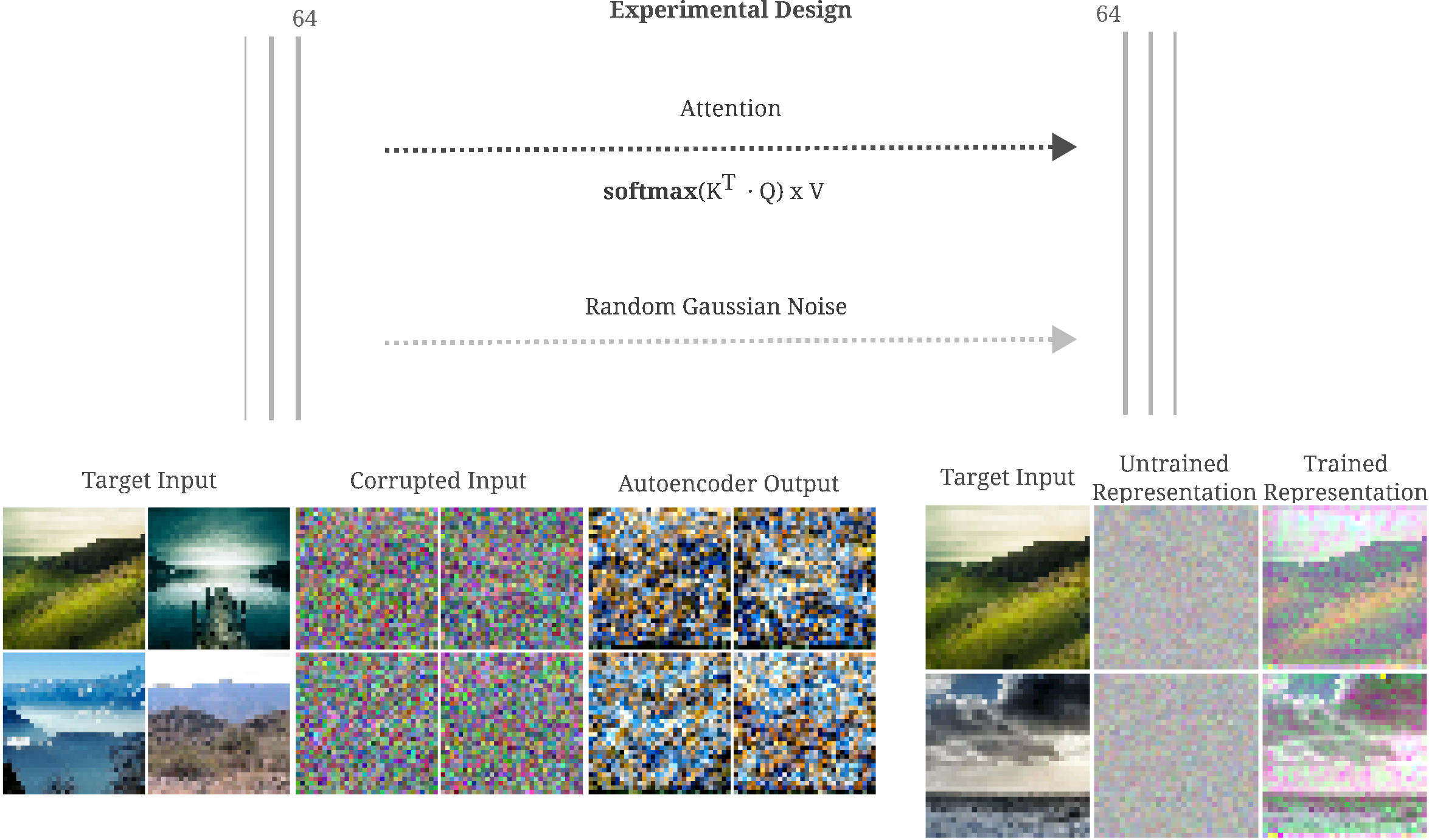In some respects, the input representation (of the output layer) of the dot-product attention autoencoder in the last figure is surprisingly good given that this module. Indeed, if we remove input and output convoltutions such that only dot-product attention transforms the input to the output, an effective autoencoder may be trained that is capable of good input representation (but does not denoise)